## Testing

### Regression

The library contains a single comprehensive regression test. For a given container type in this library, the test creates an object of the container type and an object of the corresponding standard type (e.g., `std::set`). It then performs a random sequence of methods with random arguments (e.g., inserts, erases, and so forth) on both objects. At each operation, the test checks the return value of the method, and optionally both compares this library's object with the standard's object as well as performing other consistency checks on this library's object (e.g., order preservation, when applicable, or node invariants, when applicable).

Additionally, the test integrally checks exception safety and resource leaks. This is done as follows. A special allocator type, written for the purpose of the test, both randomly throws an exceptions when allocations are performed, and tracks allocations and de-allocations. The exceptions thrown at allocations simulate memory-allocation failures; the tracking mechanism checks for memory-related bugs (e.g., resource leaks and multiple de-allocations). Both this library's containers and the containers' value-types are configured to use this allocator.

For granularity, the test is split into the several sources, each checking only some containers.

For more details, consult the files in `testsuite/ext/pb_ds/regression`.

### Performance

#### Hash-Based

##### Text `find`

###### Description

This test inserts a number of values with keys from an arbitrary text ([biblio.wickland96thirty]) into a container, then performs a series of finds using `find` . It measures the average time for `find` as a function of the number of values inserted.

It uses the test file: `performance/ext/pb_ds/text_find_timing_test.cc`

And uses the data file: `filethirty_years_among_the_dead_preproc.txt`

The test checks the effect of different range-hashing functions, trigger policies, and cache-hashing policies.

###### Results

The graphic below show the results for the native and collision-chaining hash types the function applied being a text find timing test using `find`.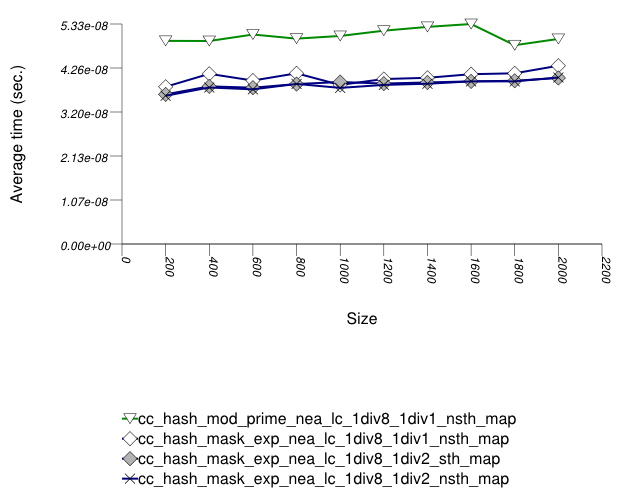The abbreviated names in the legend of the graphic above are instantiated with the types in the following table.

Name/Instantiating TypeParameterDetailsParameterDetails
n_hash_map_ncah
`std::tr1::unordered_map` `cache_hash_code` `false`
cc_hash_mod_prime_1div1_nsth_map
`cc_hash_table` `Comb_Hash_Fn` `direct_mod_range_hashing`
`Resize_Policy` `hash_standard_resize_policy` `Size_Policy` `hash_prime_size_policy`
`Trigger_Policy` `hash_load_check_resize_trigger` with αmin = 1/8 and αmax = 1/1
``` cc_hash_table ``` `Comb_Hash_Fn` `direct_mask_range_hashing`
`Resize_Policy` `hash_standard_resize_policy` `Size_Policy` `hash_exponential_size_policy`
`Trigger_Policy` `hash_load_check_resize_trigger` with αmin = 1/8 and αmax = 1/2
`cc_hash_table` `Comb_Hash_Fn` `direct_mask_range_hashing`
`Resize_Policy` `hash_standard_resize_policy` `Size_Policy` `hash_exponential_size_policy`
`Trigger_Policy` `hash_load_check_resize_trigger` with αmin = 1/8 and αmax = 1/1
`cc_hash_table` `Comb_Hash_Fn` `direct_mask_range_hashing`
`Resize_Policy` `hash_standard_resize_policy` `Size_Policy` `hash_exponential_size_policy`
`Trigger_Policy` `hash_load_check_resize_trigger` with αmin = 1/8 and αmax = 1/2
###### Observations

In this setting, the range-hashing scheme affects performance more than other policies. As the results show, containers using mod-based range-hashing (including the native hash-based container, which is currently hard-wired to this scheme) have lower performance than those using mask-based range-hashing. A modulo-based range-hashing scheme's main benefit is that it takes into account all hash-value bits. Standard string hash-functions are designed to create hash values that are nearly-uniform as is ([biblio.knuth98sorting]).

Trigger policies, i.e. the load-checks constants, affect performance to a lesser extent.

Perhaps surprisingly, storing the hash value alongside each entry affects performance only marginally, at least in this library's implementation. (Unfortunately, it was not possible to run the tests with `std::tr1::unordered_map` 's `cache_hash_code = true` , as it appeared to malfuntion.)

##### Integer `find`

###### Description

This test inserts a number of values with uniform integer keys into a container, then performs a series of finds using `find`. It measures the average time for `find` as a function of the number of values inserted.

It uses the test file: `performance/ext/pb_ds/random_int_find_timing.cc`

The test checks the effect of different underlying hash-tables, range-hashing functions, and trigger policies.

###### Results

There are two sets of results for this type, one for collision-chaining hashes, and one for general-probe hashes.

The first graphic below shows the results for the native and collision-chaining hash types. The function applied being a random integer timing test using `find`.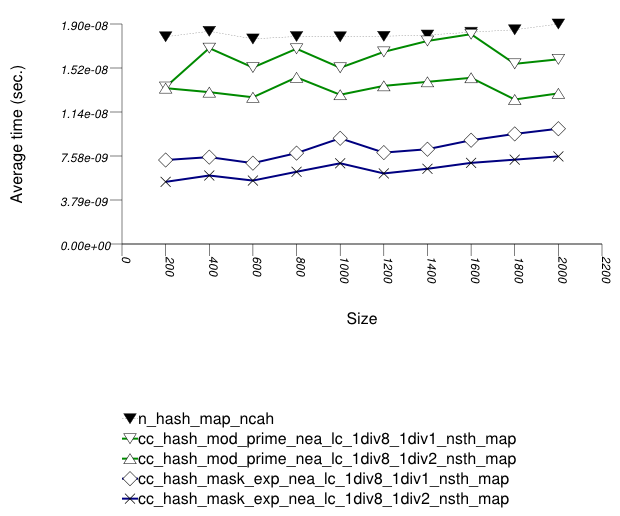The abbreviated names in the legend of the graphic above are instantiated with the types in the following table.

Name/Instantiating TypeParameterDetailsParameterDetails
n_hash_map_ncah
`std::tr1::unordered_map` `cache_hash_code` `false`
cc_hash_mod_prime_1div1_nsth_map
`cc_hash_table` `Comb_Hash_Fn` `direct_mod_range_hashing`
`Resize_Policy` `hash_standard_resize_policy` `Size_Policy` `hash_prime_size_policy`
`Trigger_Policy` `hash_load_check_resize_trigger` with αmin = 1/8 and αmax = 1/1
cc_hash_mod_prime_1div2_nsth_map
``` cc_hash_table ``` `Comb_Hash_Fn` `direct_mod_range_hashing`
`Resize_Policy` `hash_standard_resize_policy` `Size_Policy` `hash_prime_size_policy`
`Trigger_Policy` `hash_load_check_resize_trigger` with αmin = 1/8 and αmax = 1/2
`cc_hash_table` `Comb_Hash_Fn` `direct_mask_range_hashing`
`Resize_Policy` `hash_standard_resize_policy` `Size_Policy` `hash_exponential_size_policy`
`Trigger_Policy` `hash_load_check_resize_trigger` with αmin = 1/8 and αmax = 1/1
`cc_hash_table` `Comb_Hash_Fn` `direct_mask_range_hashing`
`Resize_Policy` `hash_standard_resize_policy` `Size_Policy` `hash_exponential_size_policy`
`Trigger_Policy` `hash_load_check_resize_trigger` with αmin = 1/8 and αmax = 1/2

And the second graphic shows the results for the native and general-probe hash types. The function applied being a random integer timing test using `find`.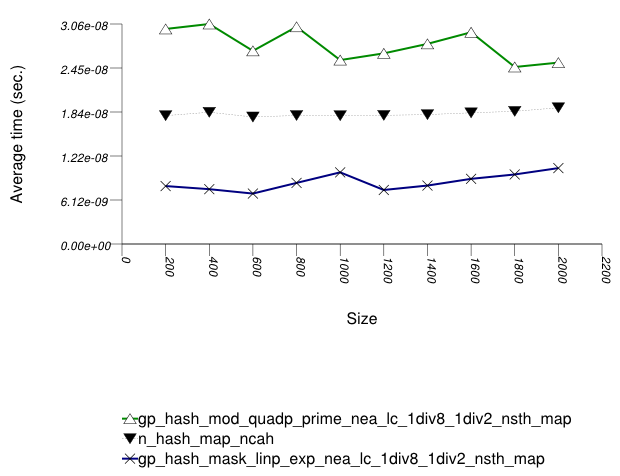The abbreviated names in the legend of the graphic above are instantiated with the types in the following table.

Name/Instantiating TypeParameterDetailsParameterDetails
n_hash_map_ncah
`std::tr1::unordered_map` `cache_hash_code` `false`
`gp_hash_table` `Comb_Hash_Fn` `direct_mod_range_hashing`
`Probe_Fn` `quadratic_probe_fn`
`Resize_Policy` `hash_standard_resize_policy` `Size_Policy` `hash_prime_size_policy`
`Trigger_Policy` `hash_load_check_resize_trigger` with αmin = 1/8 and αmax = 1/2
``` gp_hash_table ``` `Comb_Hash_Fn` `direct_mask_range_hashing`
`Probe_Fn` `linear_probe_fn`
`Resize_Policy` `hash_standard_resize_policy` `Size_Policy` `hash_exponential_size_policy`
`Trigger_Policy` `hash_load_check_resize_trigger` with αmin = 1/8 and αmax = 1/2
###### Observations

In this setting, the choice of underlying hash-table affects performance most, then the range-hashing scheme and, only finally, other policies.

When comparing probing and chaining containers, it is apparent that the probing containers are less efficient than the collision-chaining containers ( `std::tr1::unordered_map` uses collision-chaining) in this case.

Hash-Based Integer Subscript Insert Timing Test shows a different case, where the situation is reversed;

Within each type of hash-table, the range-hashing scheme affects performance more than other policies; Hash-Based Text `find` Find Timing Test also shows this. In the above graphics should be noted that `std::tr1::unordered_map` are hard-wired currently to mod-based schemes.

##### Integer Subscript `find`

###### Description

This test inserts a number of values with uniform integer keys into a container, then performs a series of finds using `operator[]`. It measures the average time for `operator[]` as a function of the number of values inserted.

It uses the test file: `performance/ext/pb_ds/random_int_subscript_find_timing.cc`

The test checks the effect of different underlying hash-tables, range-hashing functions, and trigger policies.

###### Results

There are two sets of results for this type, one for collision-chaining hashes, and one for general-probe hashes.

The first graphic below shows the results for the native and collision-chaining hash types, using as the function applied an integer subscript timing test with `find`.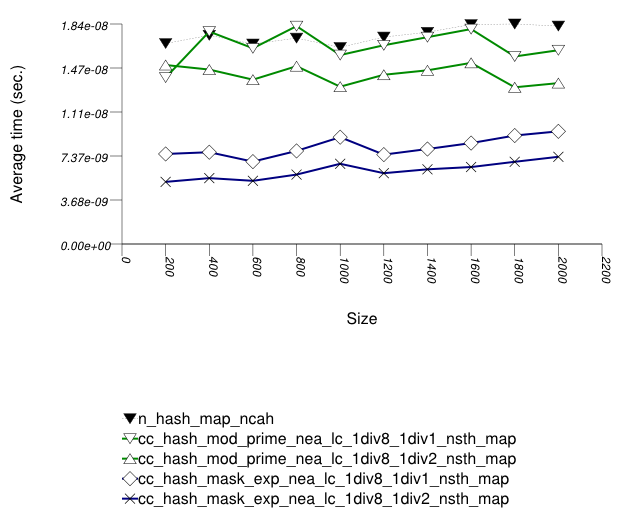The abbreviated names in the legend of the graphic above are instantiated with the types in the following table.

Name/Instantiating TypeParameterDetailsParameterDetails
n_hash_map_ncah
`std::tr1::unordered_map` `cache_hash_code` `false`
cc_hash_mod_prime_1div1_nsth_map
`cc_hash_table` `Comb_Hash_Fn` `direct_mod_range_hashing`
`Resize_Policy` `hash_standard_resize_policy` `Size_Policy` `hash_prime_size_policy`
`Trigger_Policy` `hash_load_check_resize_trigger` with αmin = 1/8 and αmax = 1/1
cc_hash_mod_prime_1div2_nsth_map
`cc_hash_table` `Comb_Hash_Fn` `direct_mod_range_hashing`
`Resize_Policy` `hash_standard_resize_policy` `Size_Policy` `hash_prime_size_policy`
`Trigger_Policy` `hash_load_check_resize_trigger` with αmin = 1/8 and αmax = 1/2
`cc_hash_table` `Comb_Hash_Fn` `direct_mask_range_hashing`
`Resize_Policy` `hash_standard_resize_policy` `Size_Policy` `hash_exponential_size_policy`
`Trigger_Policy` `hash_load_check_resize_trigger` with αmin = 1/8 and αmax = 1/1
`cc_hash_table` `Comb_Hash_Fn` `direct_mask_range_hashing`
`Resize_Policy` `hash_standard_resize_policy` `Size_Policy` `hash_exponential_size_policy`
`Trigger_Policy` `hash_load_check_resize_trigger` with αmin = 1/8 and αmax = 1/2

And the second graphic shows the results for the native and general-probe hash types. The function applied being a random integer timing test using `find`.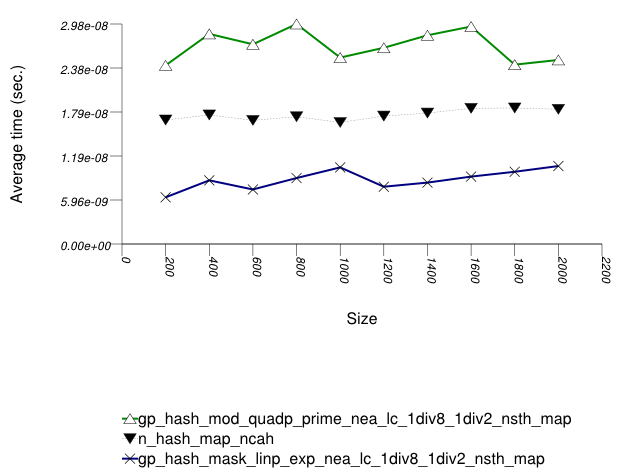The abbreviated names in the legend of the graphic above are instantiated with the types in the following table.

Name/Instantiating TypeParameterDetailsParameterDetails
n_hash_map_ncah
`std::tr1::unordered_map` `cache_hash_code` `false`
`gp_hash_table` `Comb_Hash_Fn` `direct_mod_range_hashing`
`Probe_Fn` `quadratic_probe_fn`
`Resize_Policy` `hash_standard_resize_policy` `Size_Policy` `hash_prime_size_policy`
`Trigger_Policy` `hash_load_check_resize_trigger` with αmin = 1/8 and αmax = 1/2
``` gp_hash_table ``` `Comb_Hash_Fn` `direct_mask_range_hashing`
`Probe_Fn` `linear_probe_fn`
`Resize_Policy` `hash_standard_resize_policy` `Size_Policy` `hash_exponential_size_policy`
`Trigger_Policy` `hash_load_check_resize_trigger` with αmin = 1/8 and αmax = 1/2
###### Observations

This test shows similar results to Hash-Based Integer `find` Find Timing test.

##### Integer Subscript `insert`

###### Description

This test inserts a number of values with uniform i.i.d. integer keys into a container, using `operator[]`. It measures the average time for `operator[]` as a function of the number of values inserted.

It uses the test file: `performance/ext/pb_ds/random_int_subscript_insert_timing.cc`

The test checks the effect of different underlying hash-tables.

###### Results

There are two sets of results for this type, one for collision-chaining hashes, and one for general-probe hashes.

The first graphic below shows the results for the native and collision-chaining hash types, using as the function applied an integer subscript timing test with `insert`.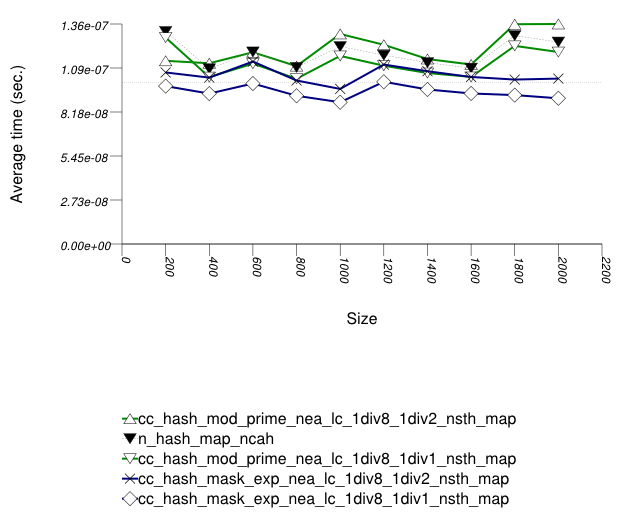The abbreviated names in the legend of the graphic above are instantiated with the types in the following table.

Name/Instantiating TypeParameterDetailsParameterDetails
n_hash_map_ncah
`std::tr1::unordered_map` `cache_hash_code` `false`
cc_hash_mod_prime_1div1_nsth_map
`cc_hash_table` `Comb_Hash_Fn` `direct_mod_range_hashing`
`Resize_Policy` `hash_standard_resize_policy` `Size_Policy` `hash_prime_size_policy`
`Trigger_Policy` `hash_load_check_resize_trigger` with αmin = 1/8 and αmax = 1/1
cc_hash_mod_prime_1div2_nsth_map
`cc_hash_table` `Comb_Hash_Fn` `direct_mod_range_hashing`
`Resize_Policy` `hash_standard_resize_policy` `Size_Policy` `hash_prime_size_policy`
`Trigger_Policy` `hash_load_check_resize_trigger` with αmin = 1/8 and αmax = 1/2
`cc_hash_table` `Comb_Hash_Fn` `direct_mask_range_hashing`
`Resize_Policy` `hash_standard_resize_policy` `Size_Policy` `hash_exponential_size_policy`
`Trigger_Policy` `hash_load_check_resize_trigger` with αmin = 1/8 and αmax = 1/1
`cc_hash_table` `Comb_Hash_Fn` `direct_mask_range_hashing`
`Resize_Policy` `hash_standard_resize_policy` `Size_Policy` `hash_exponential_size_policy`
`Trigger_Policy` `hash_load_check_resize_trigger` with αmin = 1/8 and αmax = 1/2

And the second graphic shows the results for the native and general-probe hash types. The function applied being a random integer timing test using `find`.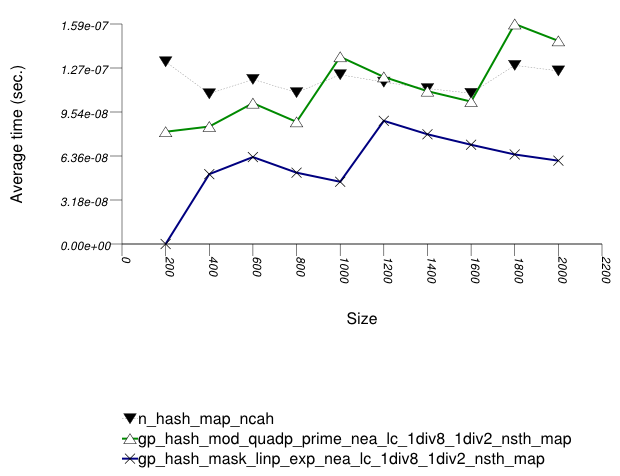The abbreviated names in the legend of the graphic above are instantiated with the types in the following table.

Name/Instantiating TypeParameterDetailsParameterDetails
n_hash_map_ncah
`std::tr1::unordered_map` `cache_hash_code` `false`
`gp_hash_table` `Comb_Hash_Fn` `direct_mod_range_hashing`
`Probe_Fn` `quadratic_probe_fn`
`Resize_Policy` `hash_standard_resize_policy` `Size_Policy` `hash_prime_size_policy`
`Trigger_Policy` `hash_load_check_resize_trigger` with αmin = 1/8 and αmax = 1/2
``` gp_hash_table ``` `Comb_Hash_Fn` `direct_mask_range_hashing`
`Probe_Fn` `linear_probe_fn`
`Resize_Policy` `hash_standard_resize_policy` `Size_Policy` `hash_exponential_size_policy`
`Trigger_Policy` `hash_load_check_resize_trigger` with αmin = 1/8 and αmax = 1/2
###### Observations

In this setting, as in Hash-Based Text `find` Find Timing test and Hash-Based Integer `find` Find Timing test , the choice of underlying hash-table underlying hash-table affects performance most, then the range-hashing scheme, and finally any other policies.

There are some differences, however:

1. In this setting, probing tables function sometimes more efficiently than collision-chaining tables. This is explained shortly.

2. The performance graphs have a "saw-tooth" shape. The average insert time rises and falls. As values are inserted into the container, the load factor grows larger. Eventually, a resize occurs. The reallocations and rehashing are relatively expensive. After this, the load factor is smaller than before.

Collision-chaining containers use indirection for greater flexibility; probing containers store values contiguously, in an array (see Figure Motivation::Different underlying data structures A and B, respectively). It follows that for simple data types, probing containers access their allocator less frequently than collision-chaining containers, (although they still have less efficient probing sequences). This explains why some probing containers fare better than collision-chaining containers in this case.

Within each type of hash-table, the range-hashing scheme affects performance more than other policies. This is similar to the situation in Hash-Based Text `find` Find Timing Test and Hash-Based Integer `find` Find Timing Test. Unsurprisingly, however, containers with lower αmax perform worse in this case, since more re-hashes are performed.

##### Integer `find` with Skewed-Distribution

###### Description

This test inserts a number of values with a markedly non-uniform integer keys into a container, then performs a series of finds using `find`. It measures the average time for `find` as a function of the number of values in the containers. The keys are generated as follows. First, a uniform integer is created. Then it is then shifted left 8 bits.

It uses the test file: `performance/ext/pb_ds/hash_zlob_random_int_find_timing.cc`

The test checks the effect of different range-hashing functions and trigger policies.

###### Results

The graphic below show the results for the native, collision-chaining, and general-probing hash types.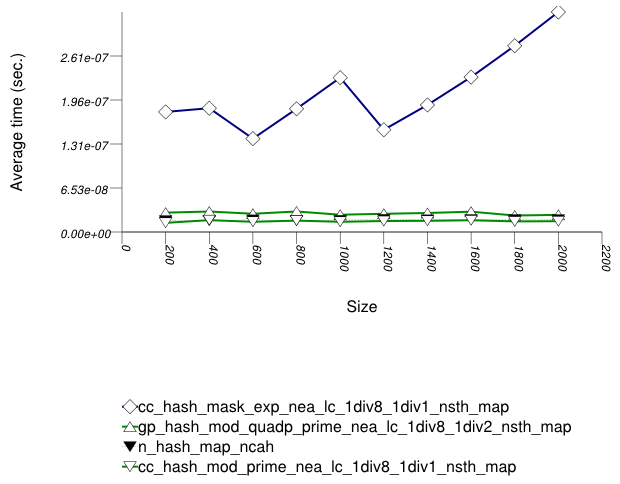The abbreviated names in the legend of the graphic above are instantiated with the types in the following table.

Name/Instantiating TypeParameterDetailsParameterDetails
n_hash_map_ncah
`std::tr1::unordered_map` `cache_hash_code` `false`
cc_hash_mod_prime_1div1_nsth_map
`cc_hash_table` `Comb_Hash_Fn` `direct_mod_range_hashing`
`Resize_Policy` `hash_standard_resize_policy` `Size_Policy` `hash_prime_size_policy`
`Trigger_Policy` `hash_load_check_resize_trigger` with αmin = 1/8 and αmax = 1/1
``` cc_hash_table ``` `Comb_Hash_Fn` `direct_mask_range_hashing`
`Resize_Policy` `hash_standard_resize_policy` `Size_Policy` `hash_exponential_size_policy`
`Trigger_Policy` `hash_load_check_resize_trigger` with αmin = 1/8 and αmax = 1/1
`gp_hash_table` `Comb_Hash_Fn` `direct_mod_range_hashing`
`Probe_Fn` `quadratic_probe_fn`
`Resize_Policy` `hash_standard_resize_policy` `Size_Policy` `hash_prime_size_policy`
`Trigger_Policy` `hash_load_check_resize_trigger` with αmin = 1/8 and αmax = 1/2
###### Observations

In this setting, the distribution of keys is so skewed that the underlying hash-table type affects performance marginally. (This is in contrast with Hash-Based Text `find` Find Timing Test, Hash-Based Integer `find` Find Timing Test, Hash-Based Integer Subscript Find Timing Test and Hash-Based Integer Subscript Insert Timing Test.)

The range-hashing scheme affects performance dramatically. A mask-based range-hashing scheme effectively maps all values into the same bucket. Access degenerates into a search within an unordered linked-list. In the graphic above, it should be noted that `std::tr1::unordered_map` is hard-wired currently to mod-based and mask-based schemes, respectively.

When observing the settings of this test, it is apparent that the keys' distribution is far from natural. One might ask if the test is not contrived to show that, in some cases, mod-based range hashing does better than mask-based range hashing. This is, in fact just the case. A more natural case in which mod-based range hashing is better was not encountered. Thus the inescapable conclusion: real-life key distributions are handled better with an appropriate hash function and a mask-based range-hashing function. (`pb_ds/example/hash_shift_mask.cc` shows an example of handling this a-priori known skewed distribution with a mask-based range-hashing function). If hash performance is bad, a χ2 test can be used to check how to transform it into a more uniform distribution.

For this reason, this library's default range-hashing function is mask-based.

##### Erase Memory Use

###### Description

This test inserts a number of uniform integer keys into a container, then erases all keys except one. It measures the final size of the container.

It uses the test file: `performance/ext/pb_ds/hash_random_int_erase_mem_usage.cc`

The test checks how containers adjust internally as their logical size decreases.

###### Results

The graphic below show the results for the native, collision-chaining, and general-probing hash types.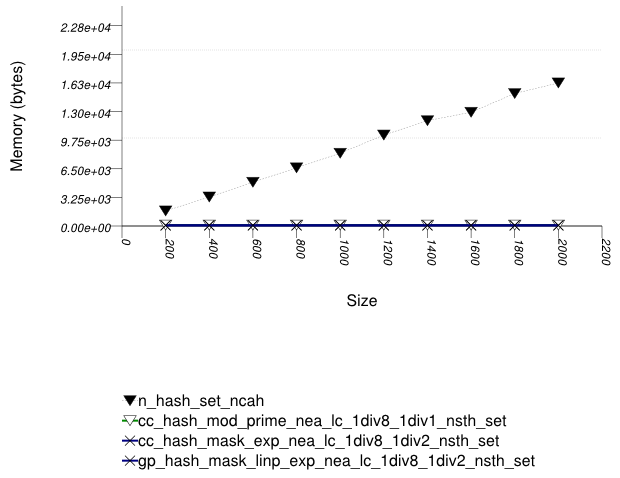The abbreviated names in the legend of the graphic above are instantiated with the types in the following table.

Name/Instantiating TypeParameterDetailsParameterDetails
n_hash_map_ncah
`std::tr1::unordered_map` `cache_hash_code` `false`
cc_hash_mod_prime_1div1_nsth_map
`cc_hash_table` `Comb_Hash_Fn` `direct_mod_range_hashing`
`Resize_Policy` `hash_standard_resize_policy` `Size_Policy` `hash_prime_size_policy`
`Trigger_Policy` `hash_load_check_resize_trigger` with αmin = 1/8 and αmax = 1/1
``` cc_hash_table ``` `Comb_Hash_Fn` `direct_mask_range_hashing`
`Resize_Policy` `hash_standard_resize_policy` `Size_Policy` `hash_exponential_size_policy`
`Trigger_Policy` `hash_load_check_resize_trigger` with αmin = 1/8 and αmax = 1/2
`gp_hash_table` `Comb_Hash_Fn` `direct_mask_range_hashing`
`Probe_Fn` `linear_probe_fn`
`Resize_Policy` `hash_standard_resize_policy` `Size_Policy` `hash_exponential_size_policy`
`Trigger_Policy` `hash_load_check_resize_trigger` with αmin = 1/8 and αmax = 1/2
###### Observations

The standard's hash-based containers act very differently than trees in this respect. When erasing numerous keys from an standard associative-container, the resulting memory user varies greatly depending on whether the container is tree-based or hash-based. This is a fundamental consequence of the standard's interface for associative containers, and it is not due to a specific implementation.

#### Branch-Based

##### Text `insert`

###### Description

This test inserts a number of values with keys from an arbitrary text ([ wickland96thirty ]) into a container using `insert` . It measures the average time for `insert` as a function of the number of values inserted.

The test checks the effect of different underlying data structures.

It uses the test file: `performance/ext/pb_ds/tree_text_insert_timing.cc`

###### Results

The three graphics below show the results for the native tree and this library's node-based trees, the native tree and this library's vector-based trees, and the native tree and this library's PATRICIA-trie, respectively.

The graphic immediately below shows the results for the native tree type and several node-based tree types.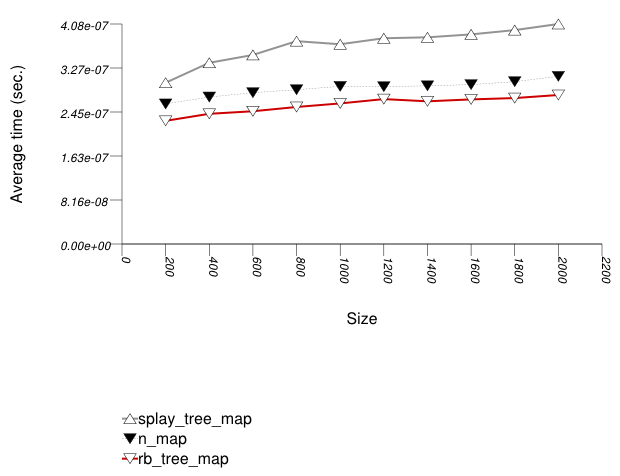The abbreviated names in the legend of the graphic above are instantiated with the types in the following table.

Name/Instantiating TypeParameterDetails
n_map
`std::map`
splay_tree_map
`tree` `Tag` `splay_tree_tag`
`Node_update` `null_node_update`
rb_tree_map
`tree` `Tag` `rb_tree_tag`
`Node_update` `null_node_update`

The graphic below shows the results for the native tree type and a vector-based tree type.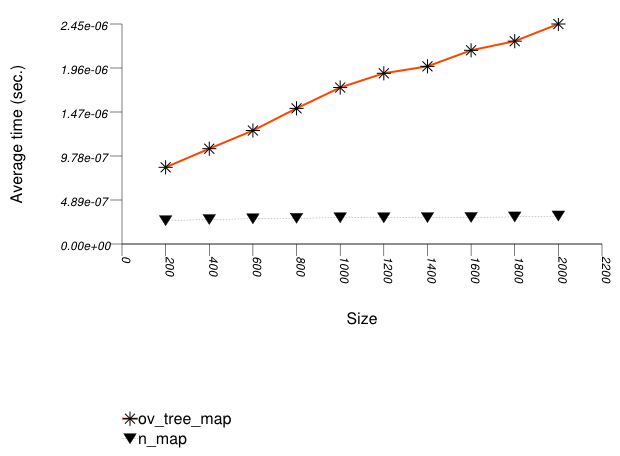The abbreviated names in the legend of the graphic above are instantiated with the types in the following table.

Name/Instantiating TypeParameterDetails
n_map
`std::map`
ov_tree_map
`tree` `Tag` `ov_tree_tag`
`Node_update` `null_node_update`

The graphic below shows the results for the native tree type and a PATRICIA trie type.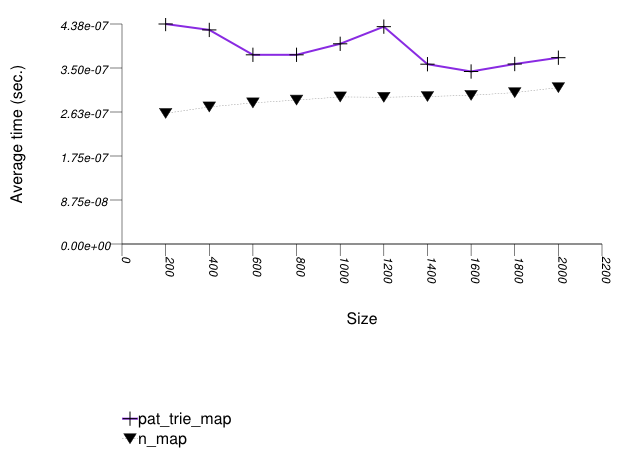The abbreviated names in the legend of the graphic above are instantiated with the types in the following table.

Name/Instantiating TypeParameterDetails
n_map
`std::map`
pat_trie_map
`tree` `Tag` `pat_trie_tag`
`Node_update` `null_node_update`
###### Observations

Observing the first graphic implies that for this setting, a splay tree (`tree` with ```Tag ``` = `splay_tree_tag`) does not do well. See also the Branch-Based Text `find` Find Timing Test. The two red-black trees perform better.

Observing the second graphic, an ordered-vector tree (`tree` with ```Tag ``` = `ov_tree_tag`) performs abysmally. Inserting into this type of tree has linear complexity [ austern00noset].

Observing the third and last graphic, A PATRICIA trie (`trie` with ```Tag ``` = `pat_trie_tag`) has abysmal performance, as well. This is not that surprising, since a large-fan-out PATRICIA trie works like a hash table with collisions resolved by a sub-trie. Each time a collision is encountered, a new "hash-table" is built A large fan-out PATRICIA trie, however, doe does well in look-ups (see Branch-Based Text `find` Find Timing Test). It may be beneficial in semi-static settings.

##### Text `find`

###### Description

This test inserts a number of values with keys from an arbitrary text ([wickland96thirty]) into a container, then performs a series of finds using `find`. It measures the average time for `find` as a function of the number of values inserted.

It uses the test file: `performance/ext/pb_ds/text_find_timing.cc`

The test checks the effect of different underlying data structures.

###### Results

The graphic immediately below shows the results for the native tree type and several other tree types.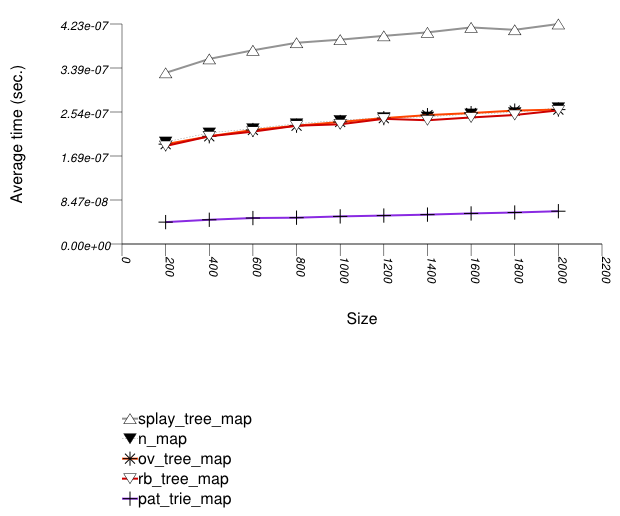The abbreviated names in the legend of the graphic above are instantiated with the types in the following table.

Name/Instantiating TypeParameterDetails
n_map
`std::map`
splay_tree_map
`tree` `Tag` `splay_tree_tag`
`Node_Update` `null_node_update`
rb_tree_map
`tree` `Tag` `rb_tree_tag`
`Node_Update` `null_node_update`
ov_tree_map
`tree` `Tag` `ov_tree_tag`
`Node_Update` `null_node_update`
pat_trie_map
`tree` `Tag` `pat_trie_tag`
`Node_Update` `null_node_update`
###### Observations

For this setting, a splay tree (`tree` with ```Tag ``` = `splay_tree_tag`) does not do well. This is possibly due to two reasons:

1. A splay tree is not guaranteed to be balanced [motwani95random]. If a splay tree contains n nodes, its average root-leaf path can be m >> log(n).

2. Assume a specific root-leaf search path has length m, and the search-target node has distance m' from the root. A red-black tree will require m + 1 comparisons to find the required node; a splay tree will require 2 m' comparisons. A splay tree, consequently, can perform many more comparisons than a red-black tree.

An ordered-vector tree (`tree` with `Tag` = `ov_tree_tag`), a red-black tree (`tree` with `Tag` = `rb_tree_tag`), and the native red-black tree all share approximately the same performance.

An ordered-vector tree is slightly slower than red-black trees, since it requires, in order to find a key, more math operations than they do. Conversely, an ordered-vector tree requires far lower space than the others. ([austern00noset], however, seems to have an implementation that is also faster than a red-black tree).

A PATRICIA trie (`trie` with `Tag` = `pat_trie_tag`) has good look-up performance, due to its large fan-out in this case. In this setting, a PATRICIA trie has look-up performance comparable to a hash table (see Hash-Based Text `find` Timing Test), but it is order preserving. This is not that surprising, since a large-fan-out PATRICIA trie works like a hash table with collisions resolved by a sub-trie. A large-fan-out PATRICIA trie does not do well on modifications (see Tree-Based and Trie-Based Text Insert Timing Test). Therefore, it is possibly beneficial in semi-static settings.

##### Text `find` with Locality-of-Reference

###### Description

This test inserts a number of values with keys from an arbitrary text ([ wickland96thirty ]) into a container, then performs a series of finds using `find`. It is different than Tree-Based and Trie-Based Text `find` Find Timing Test in the sequence of finds it performs: this test performs multiple `find`s on the same key before moving on to the next key. It measures the average time for `find` as a function of the number of values inserted.

It uses the test file: `performance/ext/pb_ds/tree_text_lor_find_timing.cc`

The test checks the effect of different underlying data structures in a locality-of-reference setting.

###### Results

The graphic immediately below shows the results for the native tree type and several other tree types.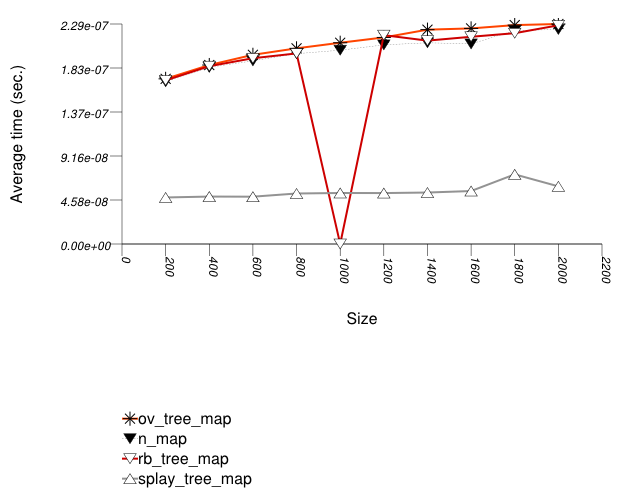The abbreviated names in the legend of the graphic above are instantiated with the types in the following table.

Name/Instantiating TypeParameterDetails
n_map
`std::map`
splay_tree_map
`tree` `Tag` `splay_tree_tag`
`Node_Update` `null_node_update`
rb_tree_map
`tree` `Tag` `rb_tree_tag`
`Node_Update` `null_node_update`
ov_tree_map
`tree` `Tag` `ov_tree_tag`
`Node_Update` `null_node_update`
pat_trie_map
`tree` `Tag` `pat_trie_tag`
`Node_Update` `null_node_update`
###### Observations

For this setting, an ordered-vector tree (`tree` with `Tag` = `ov_tree_tag`), a red-black tree (`tree` with `Tag` = `rb_tree_tag`), and the native red-black tree all share approximately the same performance.

A splay tree (`tree` with `Tag` = `splay_tree_tag`) does much better, since each (successful) find "bubbles" the corresponding node to the root of the tree.

##### `split` and `join`

###### Description

This test a container, inserts into a number of values, splits the container at the median, and joins the two containers. (If the containers are one of this library's trees, it splits and joins with the `split` and `join` method; otherwise, it uses the `erase` and `insert` methods.) It measures the time for splitting and joining the containers as a function of the number of values inserted.

It uses the test file: `performance/ext/pb_ds/tree_split_join_timing.cc`

The test checks the performance difference of `join` as opposed to a sequence of `insert` operations; by implication, this test checks the most efficient way to erase a sub-sequence from a tree-like-based container, since this can always be performed by a small sequence of splits and joins.

###### Results

The graphic immediately below shows the results for the native tree type and several other tree types.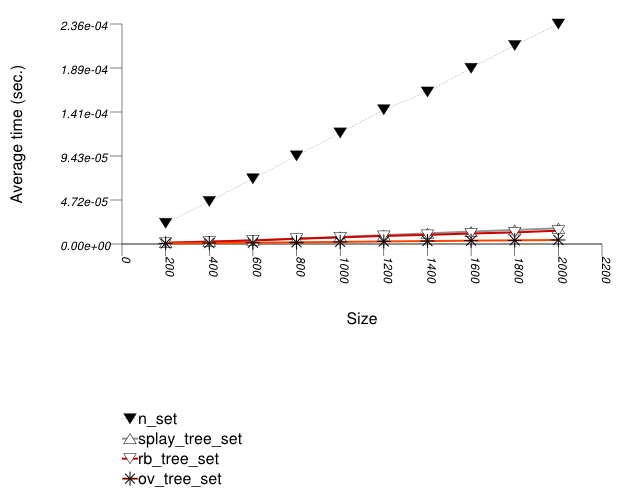The abbreviated names in the legend of the graphic above are instantiated with the types in the following table.

Name/Instantiating TypeParameterDetails
n_set
`std::set`
splay_tree_set
`tree` `Tag` `splay_tree_tag`
`Node_Update` `null_node_update`
rb_tree_set
`tree` `Tag` `rb_tree_tag`
`Node_Update` `null_node_update`
ov_tree_set
`tree` `Tag` `ov_tree_tag`
`Node_Update` `null_node_update`
pat_trie_map
`tree` `Tag` `pat_trie_tag`
`Node_Update` `null_node_update`
###### Observations

In this test, the native red-black trees must be split and joined externally, through a sequence of `erase` and `insert` operations. This is clearly super-linear, and it is not that surprising that the cost is high.

This library's tree-based containers use in this test the `split` and `join` methods, which have lower complexity: the `join` method of a splay tree (`tree` with `Tag ` = `splay_tree_tag`) is quadratic in the length of the longest root-leaf path, and linear in the total number of elements; the `join` method of a red-black tree (`tree` with `Tag ` = `rb_tree_tag`) or an ordered-vector tree (`tree` with `Tag ` = `ov_tree_tag`) is linear in the number of elements.

Asides from orders of growth, this library's trees access their allocator very little in these operations, and some of them do not access it at all. This leads to lower constants in their complexity, and, for some containers, to exception-free splits and joins (which can be determined via `container_traits`).

It is important to note that `split` and `join` are not esoteric methods - they are the most efficient means of erasing a contiguous range of values from a tree based container.

##### Order-Statistics

###### Description

This test creates a container, inserts random integers into the the container, and then checks the order-statistics of the container's values. (If the container is one of this library's trees, it does this with the `order_of_key` method of `tree_order_statistics_node_update` ; otherwise, it uses the `find` method and `std::distance`.) It measures the average time for such queries as a function of the number of values inserted.

It uses the test file: `performance/ext/pb_ds/tree_order_statistics_timing.cc`

The test checks the performance difference of policies based on node-invariant as opposed to a external functions.

###### Results

The graphic immediately below shows the results for the native tree type and several other tree types.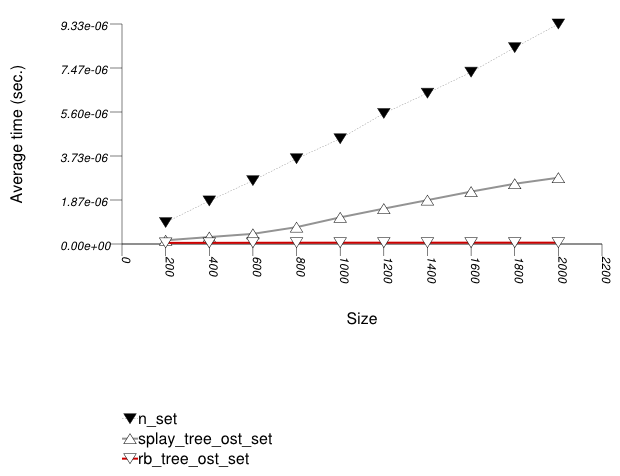The abbreviated names in the legend of the graphic above are instantiated with the types in the following table.

Name/Instantiating TypeParameterDetails
n_set
`std::set`
splay_tree_ost_set
`tree` `Tag` `splay_tree_tag`
`Node_Update` `tree_order_statistics_node_update`
rb_tree_ost_set
`tree` `Tag` `rb_tree_tag`
`Node_Update` `tree_order_statistics_node_update`
###### Observations

In this test, the native red-black tree can support order-statistics queries only externally, by performing a `find` (alternatively, `lower_bound` or `upper_bound` ) and then using `std::distance` . This is clearly linear, and it is not that surprising that the cost is high.

This library's tree-based containers use in this test the `order_of_key` method of `tree_order_statistics_node_update`. This method has only linear complexity in the length of the root-node path. Unfortunately, the average path of a splay tree (`tree` with `Tag =` `splay_tree_tag` ) can be higher than logarithmic; the longest path of a red-black tree (`tree` with `Tag =` `rb_tree_tag` ) is logarithmic in the number of elements. Consequently, the splay tree has worse performance than the red-black tree.

#### Multimap

##### Text `find` with Small Secondary-to-Primary Key Ratios

###### Description

This test inserts a number of pairs into a container. The first item of each pair is a string from an arbitrary text [wickland96thirty], and the second is a uniform i.i.d.integer. The container is a "multimap" - it considers the first member of each pair as a primary key, and the second member of each pair as a secondary key (see Motivation::Associative Containers::Alternative to Multiple Equivalent Keys). There are 400 distinct primary keys, and the ratio of secondary keys to primary keys ranges from 1 to 5.

The test measures the average find-time as a function of the number of values inserted. For this library's containers, it finds the secondary key from a container obtained from finding a primary key. For the native multimaps, it searches a range obtained using `std::equal_range` on a primary key.

It uses the test file: `performance/ext/pb_ds/multimap_text_find_timing_small.cc`

The test checks the find-time scalability of different "multimap" designs.

###### Results

The graphic below show the results for "multimaps" which use a tree-based container for primary keys.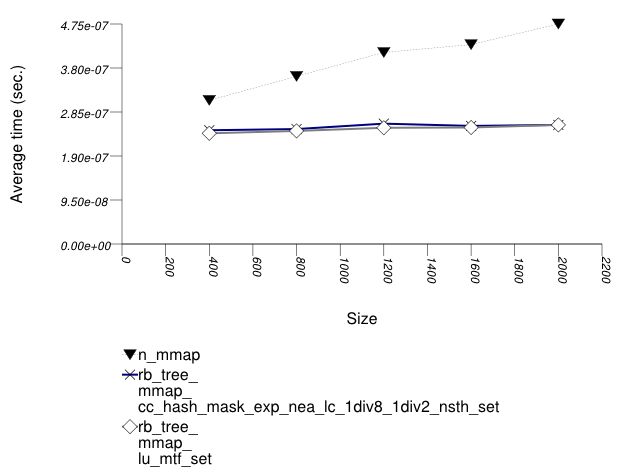The abbreviated names in the legend of the graphic above are instantiated with the types in the following table.

Name/Instantiating TypeParameterDetailsParameterDetailsParameterDetails
n_mmap
`std::multimap`
rb_tree_mmap_lu_mtf_set
`tree` `Tag` `rb_tree_tag`
`Node_Update` `null_node_update`
`Mapped` `list_update` `Update_Policy` `lu_move_to_front_policy`
`tree` `Tag` `rb_tree_tag`
`Node_Update` `null_node_update`
`Mapped` `cc_hash_table` `Comb_Hash_Fn` `direct_mask_range_hashing`
`Resize_Policy` `hash_standard_resize_policy` `Size_Policy` `hash_exponential_size_policy`
`Trigger_Policy` `hash_load_check_resize_trigger` with αmin = 1/8 and αmax = 1/2

The graphic below show the results for "multimaps" which use a hash-based container for primary keys.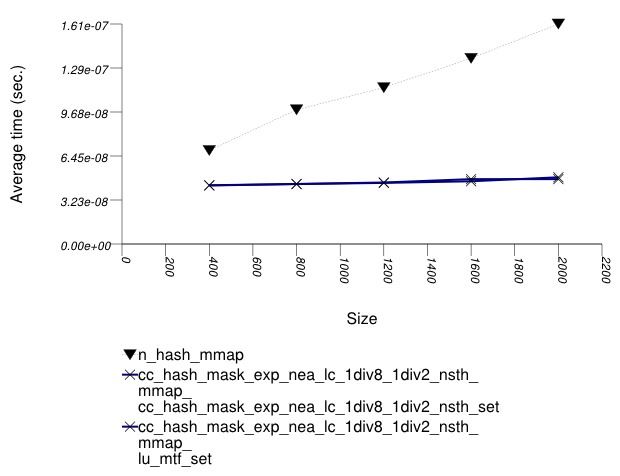The abbreviated names in the legend of the graphic above are instantiated with the types in the following table.

Name/Instantiating TypeParameterDetailsParameterDetailsParameterDetails
n_hash_mmap
`std::tr1::unordered_multimap`
rb_tree_mmap_lu_mtf_set
``` cc_hash_table ``` `Comb_Hash_Fn` `direct_mask_range_hashing`
`Resize_Policy` `hash_standard_resize_policy` `Size_Policy` `hash_exponential_size_policy`
`Trigger_Policy` `hash_load_check_resize_trigger` with αmin = 1/8 and αmax = 1/2
`Mapped` `list_update` `Update_Policy` `lu_move_to_front_policy`
``` cc_hash_table ``` `Comb_Hash_Fn` `direct_mask_range_hashing`
`Resize_Policy` `hash_standard_resize_policy` `Size_Policy` `hash_exponential_size_policy`
`Trigger_Policy` `hash_load_check_resize_trigger` with αmin = 1/8 and αmax = 1/2
`Mapped` `cc_hash_table` `Comb_Hash_Fn` `direct_mask_range_hashing`
`Resize_Policy` `hash_standard_resize_policy` `Size_Policy` `hash_exponential_size_policy`
`Trigger_Policy` `hash_load_check_resize_trigger` with αmin = 1/8 and αmax = 1/2
###### Observations

See Observations::Mapping-Semantics Considerations.

##### Text `find` with Large Secondary-to-Primary Key Ratios

###### Description

This test inserts a number of pairs into a container. The first item of each pair is a string from an arbitrary text [wickland96thirty], and the second is a uniform integer. The container is a "multimap" - it considers the first member of each pair as a primary key, and the second member of each pair as a secondary key. There are 400 distinct primary keys, and the ratio of secondary keys to primary keys ranges from 1 to 5.

The test measures the average find-time as a function of the number of values inserted. For this library's containers, it finds the secondary key from a container obtained from finding a primary key. For the native multimaps, it searches a range obtained using `std::equal_range` on a primary key.

It uses the test file: `performance/ext/pb_ds/multimap_text_find_timing_large.cc`

The test checks the find-time scalability of different "multimap" designs.

###### Results

The graphic below show the results for "multimaps" which use a tree-based container for primary keys.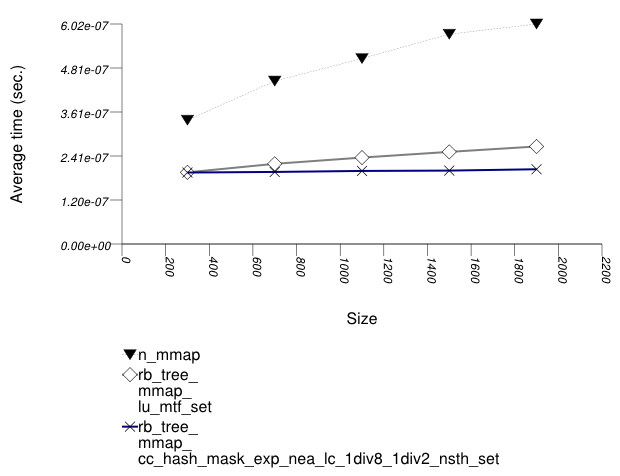The abbreviated names in the legend of the graphic above are instantiated with the types in the following table.

Name/Instantiating TypeParameterDetailsParameterDetailsParameterDetails
n_mmap
`std::multimap`
rb_tree_mmap_lu_mtf_set
`tree` `Tag` `rb_tree_tag`
`Node_Update` `null_node_update`
`Mapped` `list_update` `Update_Policy` `lu_move_to_front_policy`
`tree` `Tag` `rb_tree_tag`
`Node_Update` `null_node_update`
`Mapped` `cc_hash_table` `Comb_Hash_Fn` `direct_mask_range_hashing`
`Resize_Policy` `hash_standard_resize_policy` `Size_Policy` `hash_exponential_size_policy`
`Trigger_Policy` `hash_load_check_resize_trigger` with αmin = 1/8 and αmax = 1/2

The graphic below show the results for "multimaps" which use a hash-based container for primary keys.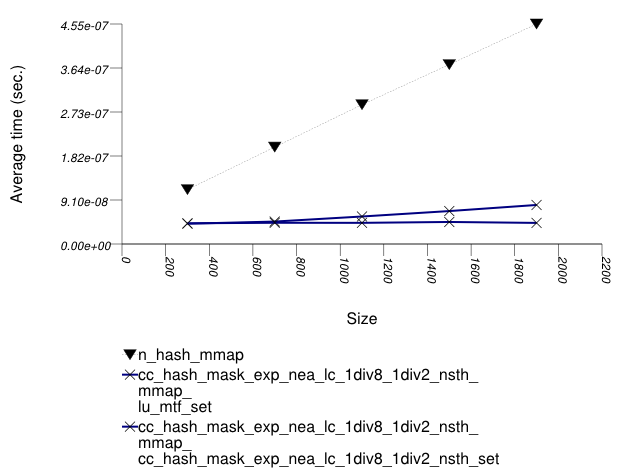The abbreviated names in the legend of the graphic above are instantiated with the types in the following table.

Name/Instantiating TypeParameterDetailsParameterDetailsParameterDetails
n_hash_mmap
`std::tr1::unordered_multimap`
rb_tree_mmap_lu_mtf_set
``` cc_hash_table ``` `Comb_Hash_Fn` `direct_mask_range_hashing`
`Resize_Policy` `hash_standard_resize_policy` `Size_Policy` `hash_exponential_size_policy`
`Trigger_Policy` `hash_load_check_resize_trigger` with αmin = 1/8 and αmax = 1/2
`Mapped` `list_update` `Update_Policy` `lu_move_to_front_policy`
``` cc_hash_table ``` `Comb_Hash_Fn` `direct_mask_range_hashing`
`Resize_Policy` `hash_standard_resize_policy` `Size_Policy` `hash_exponential_size_policy`
`Trigger_Policy` `hash_load_check_resize_trigger` with αmin = 1/8 and αmax = 1/2
`Mapped` `cc_hash_table` `Comb_Hash_Fn` `direct_mask_range_hashing`
`Resize_Policy` `hash_standard_resize_policy` `Size_Policy` `hash_exponential_size_policy`
`Trigger_Policy` `hash_load_check_resize_trigger` with αmin = 1/8 and αmax = 1/2
###### Observations

See Observations::Mapping-Semantics Considerations.

##### Text `insert` with Small Secondary-to-Primary Key Ratios

###### Description

This test inserts a number of pairs into a container. The first item of each pair is a string from an arbitrary text [wickland96thirty], and the second is a uniform integer. The container is a "multimap" - it considers the first member of each pair as a primary key, and the second member of each pair as a secondary key. There are 400 distinct primary keys, and the ratio of secondary keys to primary keys ranges from 1 to 5.

The test measures the average insert-time as a function of the number of values inserted. For this library's containers, it inserts a primary key into the primary associative container, then a secondary key into the secondary associative container. For the native multimaps, it obtains a range using `std::equal_range`, and inserts a value only if it was not contained already.

It uses the test file: `performance/ext/pb_ds/multimap_text_insert_timing_small.cc`

The test checks the insert-time scalability of different "multimap" designs.

###### Results

The graphic below show the results for "multimaps" which use a tree-based container for primary keys.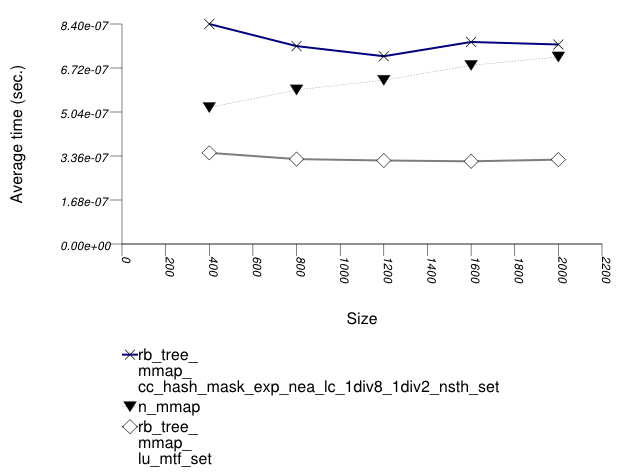The abbreviated names in the legend of the graphic above are instantiated with the types in the following table.

Name/Instantiating TypeParameterDetailsParameterDetailsParameterDetails
n_mmap
`std::multimap`
rb_tree_mmap_lu_mtf_set
`tree` `Tag` `rb_tree_tag`
`Node_Update` `null_node_update`
`Mapped` `list_update` `Update_Policy` `lu_move_to_front_policy`
`tree` `Tag` `rb_tree_tag`
`Node_Update` `null_node_update`
`Mapped` `cc_hash_table` `Comb_Hash_Fn` `direct_mask_range_hashing`
`Resize_Policy` `hash_standard_resize_policy` `Size_Policy` `hash_exponential_size_policy`
`Trigger_Policy` `hash_load_check_resize_trigger` with αmin = 1/8 and αmax = 1/2

The graphic below show the results for "multimaps" which use a hash-based container for primary keys.The abbreviated names in the legend of the graphic above are instantiated with the types in the following table.

Name/Instantiating TypeParameterDetailsParameterDetailsParameterDetails
n_hash_mmap
`std::tr1::unordered_multimap`
rb_tree_mmap_lu_mtf_set
``` cc_hash_table ``` `Comb_Hash_Fn` `direct_mask_range_hashing`
`Resize_Policy` `hash_standard_resize_policy` `Size_Policy` `hash_exponential_size_policy`
`Trigger_Policy` `hash_load_check_resize_trigger` with αmin = 1/8 and αmax = 1/2
`Mapped` `list_update` `Update_Policy` `lu_move_to_front_policy`
``` cc_hash_table ``` `Comb_Hash_Fn` `direct_mask_range_hashing`
`Resize_Policy` `hash_standard_resize_policy` `Size_Policy` `hash_exponential_size_policy`
`Trigger_Policy` `hash_load_check_resize_trigger` with αmin = 1/8 and αmax = 1/2
`Mapped` `cc_hash_table` `Comb_Hash_Fn` `direct_mask_range_hashing`
`Resize_Policy` `hash_standard_resize_policy` `Size_Policy` `hash_exponential_size_policy`
`Trigger_Policy` `hash_load_check_resize_trigger` with αmin = 1/8 and αmax = 1/2
###### Observations

See Observations::Mapping-Semantics Considerations.

##### Text `insert` with Small Secondary-to-Primary Key Ratios

###### Description

This test inserts a number of pairs into a container. The first item of each pair is a string from an arbitrary text [wickland96thirty], and the second is a uniform integer. The container is a "multimap" - it considers the first member of each pair as a primary key, and the second member of each pair as a secondary key. There are 400 distinct primary keys, and the ratio of secondary keys to primary keys ranges from 1 to 5.

The test measures the average insert-time as a function of the number of values inserted. For this library's containers, it inserts a primary key into the primary associative container, then a secondary key into the secondary associative container. For the native multimaps, it obtains a range using `std::equal_range`, and inserts a value only if it was not contained already.

It uses the test file: `performance/ext/pb_ds/multimap_text_insert_timing_large.cc`

The test checks the insert-time scalability of different "multimap" designs.

###### Results

The graphic below show the results for "multimaps" which use a tree-based container for primary keys.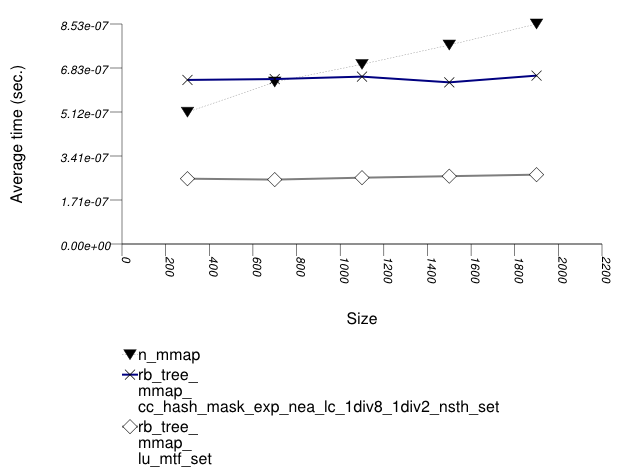The abbreviated names in the legend of the graphic above are instantiated with the types in the following table.

Name/Instantiating TypeParameterDetailsParameterDetailsParameterDetails
n_mmap
`std::multimap`
rb_tree_mmap_lu_mtf_set
`tree` `Tag` `rb_tree_tag`
`Node_Update` `null_node_update`
`Mapped` `list_update` `Update_Policy` `lu_move_to_front_policy`
`tree` `Tag` `rb_tree_tag`
`Node_Update` `null_node_update`
`Mapped` `cc_hash_table` `Comb_Hash_Fn` `direct_mask_range_hashing`
`Resize_Policy` `hash_standard_resize_policy` `Size_Policy` `hash_exponential_size_policy`
`Trigger_Policy` `hash_load_check_resize_trigger` with αmin = 1/8 and αmax = 1/2

The graphic below show the results for "multimaps" which use a hash-based container for primary keys.The abbreviated names in the legend of the graphic above are instantiated with the types in the following table.

Name/Instantiating TypeParameterDetailsParameterDetailsParameterDetails
n_hash_mmap
`std::tr1::unordered_multimap`
rb_tree_mmap_lu_mtf_set
``` cc_hash_table ``` `Comb_Hash_Fn` `direct_mask_range_hashing`
`Resize_Policy` `hash_standard_resize_policy` `Size_Policy` `hash_exponential_size_policy`
`Trigger_Policy` `hash_load_check_resize_trigger` with αmin = 1/8 and αmax = 1/2
`Mapped` `list_update` `Update_Policy` `lu_move_to_front_policy`
``` cc_hash_table ``` `Comb_Hash_Fn` `direct_mask_range_hashing`
`Resize_Policy` `hash_standard_resize_policy` `Size_Policy` `hash_exponential_size_policy`
`Trigger_Policy` `hash_load_check_resize_trigger` with αmin = 1/8 and αmax = 1/2
`Mapped` `cc_hash_table` `Comb_Hash_Fn` `direct_mask_range_hashing`
`Resize_Policy` `hash_standard_resize_policy` `Size_Policy` `hash_exponential_size_policy`
`Trigger_Policy` `hash_load_check_resize_trigger` with αmin = 1/8 and αmax = 1/2
###### Observations

See Observations::Mapping-Semantics Considerations.

##### Text `insert` with Small Secondary-to-Primary Key Ratios Memory Use

###### Description

This test inserts a number of pairs into a container. The first item of each pair is a string from an arbitrary text [wickland96thirty], and the second is a uniform integer. The container is a "multimap" - it considers the first member of each pair as a primary key, and the second member of each pair as a secondary key. There are 100 distinct primary keys, and the ratio of secondary keys to primary keys ranges to about 20.

The test measures the memory use as a function of the number of values inserted.

It uses the test file: `performance/ext/pb_ds/multimap_text_insert_mem_usage_small.cc`

The test checks the memory scalability of different "multimap" designs.

###### Results

The graphic below show the results for "multimaps" which use a tree-based container for primary keys.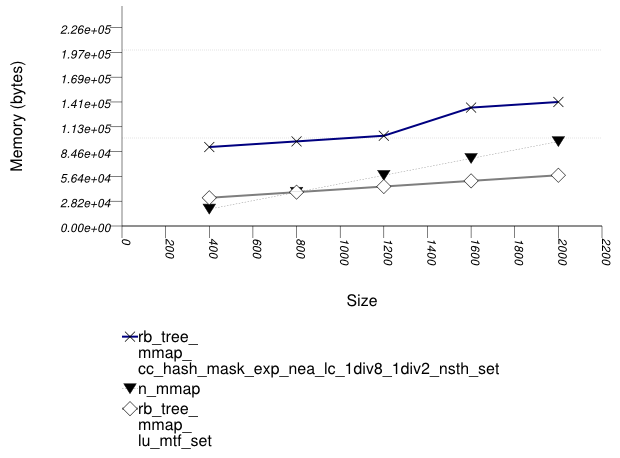The abbreviated names in the legend of the graphic above are instantiated with the types in the following table.

Name/Instantiating TypeParameterDetailsParameterDetailsParameterDetails
n_mmap
`std::multimap`
rb_tree_mmap_lu_mtf_set
`tree` `Tag` `rb_tree_tag`
`Node_Update` `null_node_update`
`Mapped` `list_update` `Update_Policy` `lu_move_to_front_policy`
`tree` `Tag` `rb_tree_tag`
`Node_Update` `null_node_update`
`Mapped` `cc_hash_table` `Comb_Hash_Fn` `direct_mask_range_hashing`
`Resize_Policy` `hash_standard_resize_policy` `Size_Policy` `hash_exponential_size_policy`
`Trigger_Policy` `hash_load_check_resize_trigger` with αmin = 1/8 and αmax = 1/2

The graphic below show the results for "multimaps" which use a hash-based container for primary keys.The abbreviated names in the legend of the graphic above are instantiated with the types in the following table.

Name/Instantiating TypeParameterDetailsParameterDetailsParameterDetails
n_hash_mmap
`std::tr1::unordered_multimap`
rb_tree_mmap_lu_mtf_set
``` cc_hash_table ``` `Comb_Hash_Fn` `direct_mask_range_hashing`
`Resize_Policy` `hash_standard_resize_policy` `Size_Policy` `hash_exponential_size_policy`
`Trigger_Policy` `hash_load_check_resize_trigger` with αmin = 1/8 and αmax = 1/2
`Mapped` `list_update` `Update_Policy` `lu_move_to_front_policy`
``` cc_hash_table ``` `Comb_Hash_Fn` `direct_mask_range_hashing`
`Resize_Policy` `hash_standard_resize_policy` `Size_Policy` `hash_exponential_size_policy`
`Trigger_Policy` `hash_load_check_resize_trigger` with αmin = 1/8 and αmax = 1/2
`Mapped` `cc_hash_table` `Comb_Hash_Fn` `direct_mask_range_hashing`
`Resize_Policy` `hash_standard_resize_policy` `Size_Policy` `hash_exponential_size_policy`
`Trigger_Policy` `hash_load_check_resize_trigger` with αmin = 1/8 and αmax = 1/2
###### Observations

See Observations::Mapping-Semantics Considerations.

##### Text `insert` with Small Secondary-to-Primary Key Ratios Memory Use

###### Description

This test inserts a number of pairs into a container. The first item of each pair is a string from an arbitrary text [wickland96thirty], and the second is a uniform integer. The container is a "multimap" - it considers the first member of each pair as a primary key, and the second member of each pair as a secondary key. There are 100 distinct primary keys, and the ratio of secondary keys to primary keys ranges to about 20.

The test measures the memory use as a function of the number of values inserted.

It uses the test file: `performance/ext/pb_ds/multimap_text_insert_mem_usage_large.cc`

The test checks the memory scalability of different "multimap" designs.

###### Results

The graphic below show the results for "multimaps" which use a tree-based container for primary keys.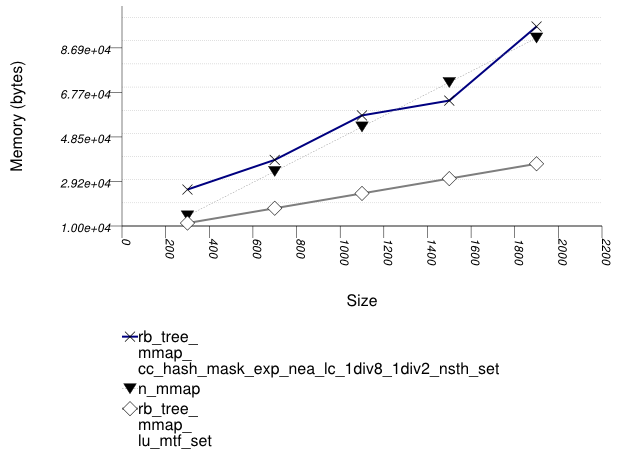The abbreviated names in the legend of the graphic above are instantiated with the types in the following table.

Name/Instantiating TypeParameterDetailsParameterDetailsParameterDetails
n_mmap
`std::multimap`
rb_tree_mmap_lu_mtf_set
`tree` `Tag` `rb_tree_tag`
`Node_Update` `null_node_update`
`Mapped` `list_update` `Update_Policy` `lu_move_to_front_policy`
`tree` `Tag` `rb_tree_tag`
`Node_Update` `null_node_update`
`Mapped` `cc_hash_table` `Comb_Hash_Fn` `direct_mask_range_hashing`
`Resize_Policy` `hash_standard_resize_policy` `Size_Policy` `hash_exponential_size_policy`
`Trigger_Policy` `hash_load_check_resize_trigger` with αmin = 1/8 and αmax = 1/2

The graphic below show the results for "multimaps" which use a hash-based container for primary keys.The abbreviated names in the legend of the graphic above are instantiated with the types in the following table.

Name/Instantiating TypeParameterDetailsParameterDetailsParameterDetails
n_hash_mmap
`std::tr1::unordered_multimap`
rb_tree_mmap_lu_mtf_set
``` cc_hash_table ``` `Comb_Hash_Fn` `direct_mask_range_hashing`
`Resize_Policy` `hash_standard_resize_policy` `Size_Policy` `hash_exponential_size_policy`
`Trigger_Policy` `hash_load_check_resize_trigger` with αmin = 1/8 and αmax = 1/2
`Mapped` `list_update` `Update_Policy` `lu_move_to_front_policy`
``` cc_hash_table ``` `Comb_Hash_Fn` `direct_mask_range_hashing`
`Resize_Policy` `hash_standard_resize_policy` `Size_Policy` `hash_exponential_size_policy`
`Trigger_Policy` `hash_load_check_resize_trigger` with αmin = 1/8 and αmax = 1/2
`Mapped` `cc_hash_table` `Comb_Hash_Fn` `direct_mask_range_hashing`
`Resize_Policy` `hash_standard_resize_policy` `Size_Policy` `hash_exponential_size_policy`
`Trigger_Policy` `hash_load_check_resize_trigger` with αmin = 1/8 and αmax = 1/2
###### Observations

See Observations::Mapping-Semantics Considerations.

#### Priority Queue

##### Text `push`

###### Description

This test inserts a number of values with keys from an arbitrary text ([ wickland96thirty ]) into a container using `push`. It measures the average time for `push` as a function of the number of values pushed.

It uses the test file: `performance/ext/pb_ds/priority_queue_text_push_timing.cc`

The test checks the effect of different underlying data structures.

###### Results

The two graphics below show the results for the native priority_queues and this library's priority_queues.

The graphic immediately below shows the results for the native priority_queue type instantiated with different underlying container types versus several different versions of library's priority_queues.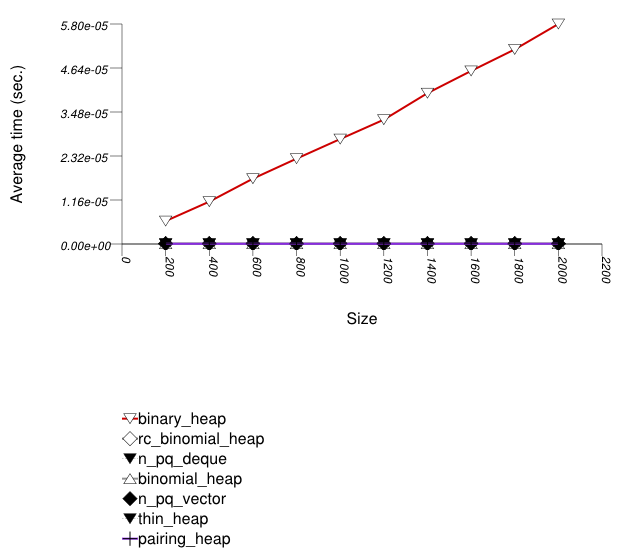The abbreviated names in the legend of the graphic above are instantiated with the types in the following table.

Name/Instantiating TypeParameterDetails
n_pq_vector
`std::priority_queue` `Sequence` `std::vector`
n_pq_deque
`std::priority_queue` `Sequence` `std::deque`
binary_heap
`priority_queue` `Tag` `binary_heap_tag`
binomial_heap
`priority_queue` `Tag` `binomial_heap_tag`
rc_binomial_heap
`priority_queue` `Tag` `rc_binomial_heap_tag`
thin_heap
`priority_queue` `Tag` `thin_heap_tag`
pairing_heap
`priority_queue` `Tag` `pairing_heap_tag`

The graphic below shows the results for the binary-heap based native priority queues and this library's pairing-heap priority_queue data structures.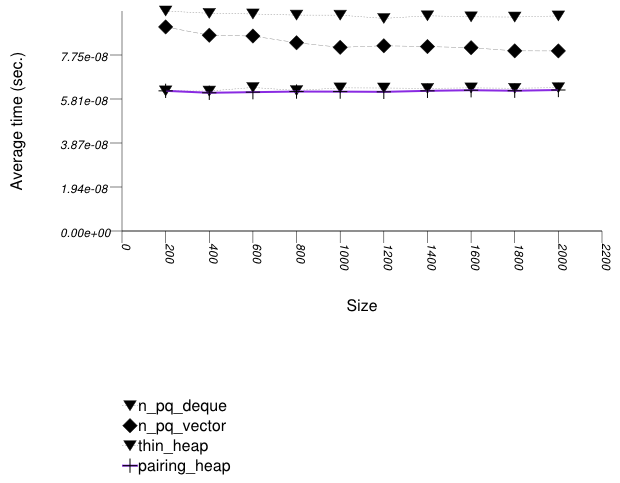The abbreviated names in the legend of the graphic above are instantiated with the types in the following table.

Name/Instantiating TypeParameterDetails
n_pq_vector
`std::priority_queue` `Sequence` `std::vector`
n_pq_deque
`std::priority_queue` `Sequence` `std::deque`
thin_heap
`priority_queue` `Tag` `thin_heap_tag`
pairing_heap
`priority_queue` `Tag` `pairing_heap_tag`
###### Observations

Pairing heaps (`priority_queue` with `Tag` = `pairing_heap_tag`) are the most suited for sequences of `push` and `pop` operations of non-primitive types (e.g. `std::string`s). (See Priority Queue Text `push` and `pop` Timing Test.) They are less constrained than binomial heaps, e.g., and since they are node-based, they outperform binary heaps. (See Priority Queue Random Integer `push` Timing Test for the case of primitive types.)

The standard's priority queues do not seem to perform well in this case: the `std::vector` implementation needs to perform a logarithmic sequence of string operations for each operation, and the deque implementation is possibly hampered by its need to manipulate a relatively-complex type (deques support a O(1) `push_front`, even though it is not used by `std::priority_queue`.)

##### Text `push` and `pop`

###### Description

This test inserts a number of values with keys from an arbitrary text ([ wickland96thirty ]) into a container using `push` , then removes them using `pop` . It measures the average time for `push` as a function of the number of values.

It uses the test file: `performance/ext/pb_ds/priority_queue_text_push_pop_timing.cc`

The test checks the effect of different underlying data structures.

###### Results

The two graphics below show the results for the native priority_queues and this library's priority_queues.

The graphic immediately below shows the results for the native priority_queue type instantiated with different underlying container types versus several different versions of library's priority_queues.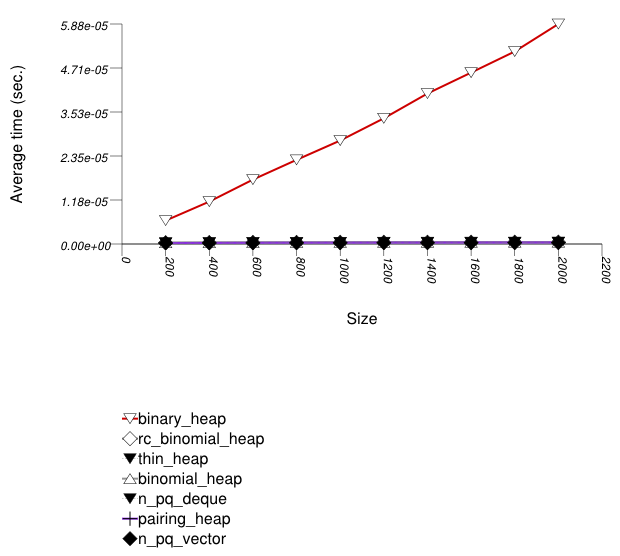The abbreviated names in the legend of the graphic above are instantiated with the types in the following table.

Name/Instantiating TypeParameterDetails
n_pq_vector
`std::priority_queue` `Sequence` `std::vector`
n_pq_deque
`std::priority_queue` `Sequence` `std::deque`
binary_heap
`priority_queue` `Tag` `binary_heap_tag`
binomial_heap
`priority_queue` `Tag` `binomial_heap_tag`
rc_binomial_heap
`priority_queue` `Tag` `rc_binomial_heap_tag`
thin_heap
`priority_queue` `Tag` `thin_heap_tag`
pairing_heap
`priority_queue` `Tag` `pairing_heap_tag`

The graphic below shows the results for the native priority queues and this library's pairing-heap priority_queue data structures.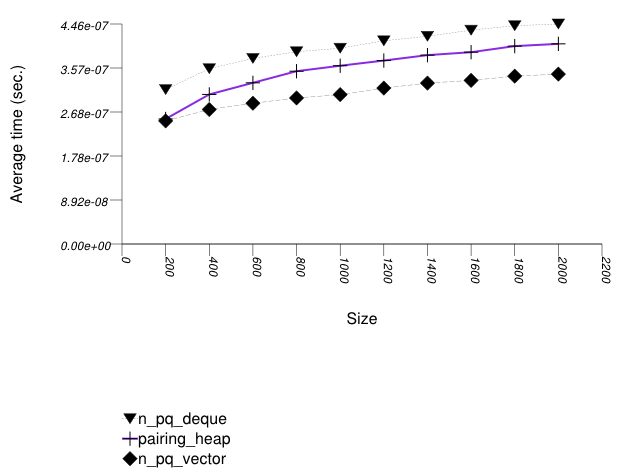The abbreviated names in the legend of the graphic above are instantiated with the types in the following table.

Name/Instantiating TypeParameterDetails
n_pq_vector
`std::priority_queue` adapting `std::vector` `Sequence` `std::vector`
n_pq_deque
`std::priority_queue` `Sequence` `std::deque`
pairing_heap
`priority_queue` `Tag` `pairing_heap_tag`
###### Observations

These results are very similar to Priority Queue Text `push` Timing Test. As stated there, pairing heaps (`priority_queue` with `Tag` = `pairing_heap_tag`) are most suited for `push` and `pop` sequences of non-primitive types such as strings. Observing these two tests, one can note that a pairing heap outperforms the others in terms of `push` operations, but equals binary heaps (`priority_queue` with `Tag` = `binary_heap_tag`) if the number of `push` and `pop` operations is equal. As the number of `pop` operations is at most equal to the number of `push` operations, pairing heaps are better in this case. See Priority Queue Random Integer `push` and `pop` Timing Test for a case which is different.

##### Integer `push`

###### Description

This test inserts a number of values with integer keys into a container using `push`. It measures the average time for `push` as a function of the number of values.

It uses the test file: `performance/ext/pb_ds/priority_queue_random_int_push_timing.cc`

The test checks the effect of different underlying data structures.

###### Results

The two graphics below show the results for the native priority_queues and this library's priority_queues.

The graphic immediately below shows the results for the native priority_queue type instantiated with different underlying container types versus several different versions of library's priority_queues.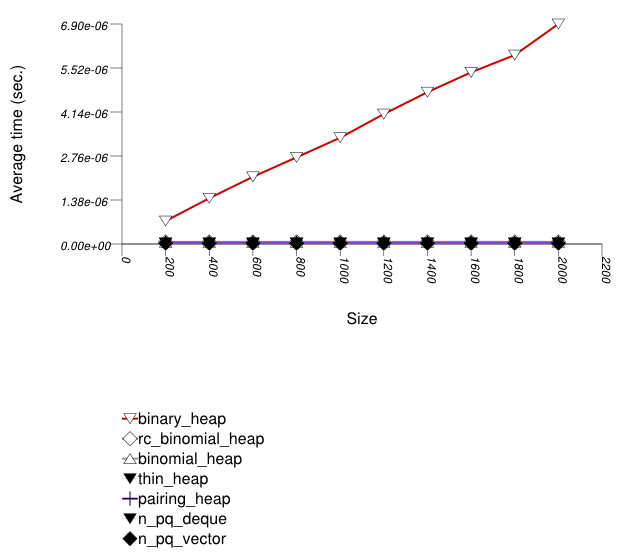The abbreviated names in the legend of the graphic above are instantiated with the types in the following table.

Name/Instantiating TypeParameterDetails
n_pq_vector
`std::priority_queue` `Sequence` `std::vector`
n_pq_deque
`std::priority_queue` `Sequence` `std::deque`
binary_heap
`priority_queue` `Tag` `binary_heap_tag`
binomial_heap
`priority_queue` `Tag` `binomial_heap_tag`
rc_binomial_heap
`priority_queue` `Tag` `rc_binomial_heap_tag`
thin_heap
`priority_queue` `Tag` `thin_heap_tag`
pairing_heap
`priority_queue` `Tag` `pairing_heap_tag`

The graphic below shows the results for the binary-heap based native priority queues and this library's priority_queue data structures.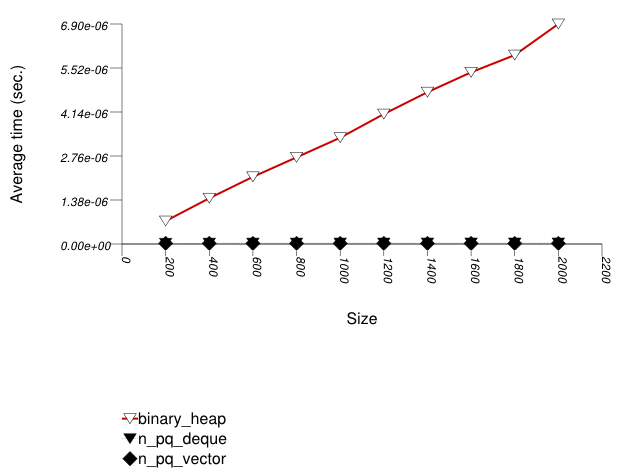The abbreviated names in the legend of the graphic above are instantiated with the types in the following table.

Name/Instantiating TypeParameterDetails
n_pq_vector
`std::priority_queue` adapting `std::vector` `Sequence` `std::vector`
n_pq_deque
`std::priority_queue` `Sequence` `std::deque`
binary_heap
`priority_queue` `Tag` `binary_heap_tag`
###### Observations

Binary heaps are the most suited for sequences of `push` and `pop` operations of primitive types (e.g. ints). They are less constrained than any other type, and since it is very efficient to store such types in arrays, they outperform even pairing heaps. (See Priority Queue Text `push` Timing Test for the case of non-primitive types.)

##### Integer `push`

###### Description

This test inserts a number of values with integer keys into a container using `push` , then removes them using `pop` . It measures the average time for `push` and `pop` as a function of the number of values.

It uses the test file: `performance/ext/pb_ds/priority_queue_random_int_push_pop_timing.cc`

The test checks the effect of different underlying data structures.

###### Results

The graphic immediately below shows the results for the native priority_queue type instantiated with different underlying container types versus several different versions of library's priority_queues.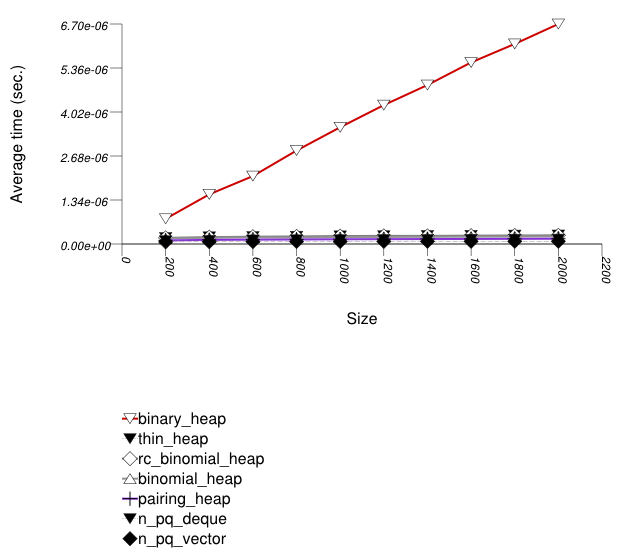The abbreviated names in the legend of the graphic above are instantiated with the types in the following table.

Name/Instantiating TypeParameterDetails
n_pq_vector
`std::priority_queue` `Sequence` `std::vector`
n_pq_deque
`std::priority_queue` `Sequence` `std::deque`
binary_heap
`priority_queue` `Tag` `binary_heap_tag`
binomial_heap
`priority_queue` `Tag` `binomial_heap_tag`
rc_binomial_heap
`priority_queue` `Tag` `rc_binomial_heap_tag`
thin_heap
`priority_queue` `Tag` `thin_heap_tag`
pairing_heap
`priority_queue` `Tag` `pairing_heap_tag`
###### Observations

Binary heaps are the most suited for sequences of `push` and `pop` operations of primitive types (e.g. ints). This is explained in Priority Queue Random Int `push` Timing Test. (See Priority Queue Text `push` Timing Test for the case of primitive types.)

At first glance it seems that the standard's vector-based priority queue is approximately on par with this library's corresponding priority queue. There are two differences however:

1. The standard's priority queue does not downsize the underlying vector (or deque) as the priority queue becomes smaller (see Priority Queue Text `pop` Memory Use Test). It is therefore gaining some speed at the expense of space.

2. From Priority Queue Random Integer `push` and `pop` Timing Test, it seems that the standard's priority queue is slower in terms of `push` operations. Since the number of `pop` operations is at most that of `push` operations, the test here is the "best" for the standard's priority queue.

##### Text `pop` Memory Use

###### Description

This test inserts a number of values with keys from an arbitrary text ([ wickland96thirty ]) into a container, then pops them until only one is left in the container. It measures the memory use as a function of the number of values pushed to the container.

It uses the test file: `performance/ext/pb_ds/priority_queue_text_pop_mem_usage.cc`

The test checks the effect of different underlying data structures.

###### Results

The graphic immediately below shows the results for the native priority_queue type instantiated with different underlying container types versus several different versions of library's priority_queues.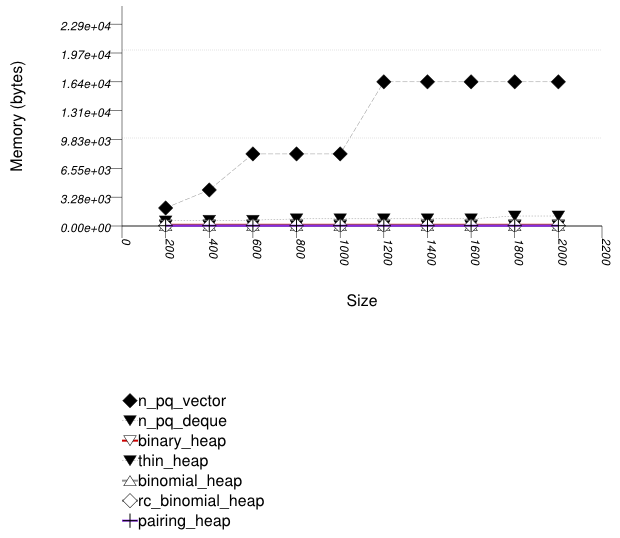The abbreviated names in the legend of the graphic above are instantiated with the types in the following table.

Name/Instantiating TypeParameterDetails
n_pq_vector
`std::priority_queue` `Sequence` `std::vector`
n_pq_deque
`std::priority_queue` `Sequence` `std::deque`
binary_heap
`priority_queue` `Tag` `binary_heap_tag`
binomial_heap
`priority_queue` `Tag` `binomial_heap_tag`
rc_binomial_heap
`priority_queue` `Tag` `rc_binomial_heap_tag`
thin_heap
`priority_queue` `Tag` `thin_heap_tag`
pairing_heap
`priority_queue` `Tag` `pairing_heap_tag`
###### Observations

The priority queue implementations (excluding the standard's) use memory proportionally to the number of values they hold: node-based implementations (e.g., a pairing heap) do so naturally; this library's binary heap de-allocates memory when a certain lower threshold is exceeded.

Note from Priority Queue Text `push` and `pop` Timing Test and Priority Queue Random Integer `push` and `pop` Timing Test that this does not impede performance compared to the standard's priority queues.

See Hash-Based Erase Memory Use Test for a similar phenomenon regarding priority queues.

##### Text `join`

###### Description

This test inserts a number of values with keys from an arbitrary text ([ wickland96thirty ]) into two containers, then merges the containers. It uses `join` for this library's priority queues; for the standard's priority queues, it successively pops values from one container and pushes them into the other. The test measures the average time as a function of the number of values.

It uses the test file: `performance/ext/pb_ds/priority_queue_text_join_timing.cc`

The test checks the effect of different underlying data structures.

###### Results

The graphic immediately below shows the results for the native priority_queue type instantiated with different underlying container types versus several different versions of library's priority_queues.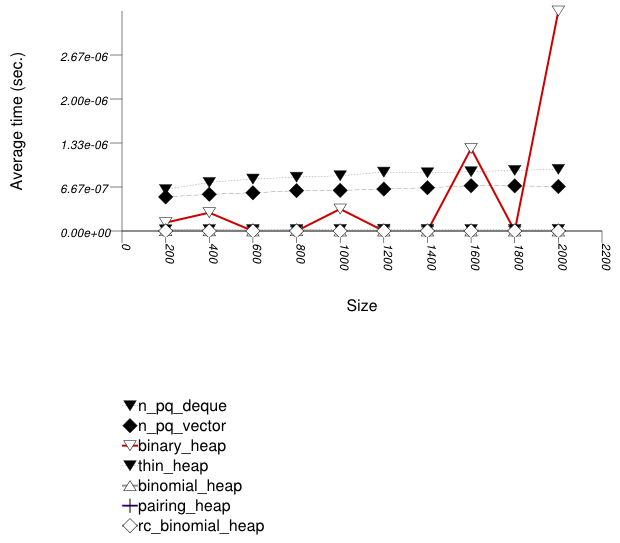The abbreviated names in the legend of the graphic above are instantiated with the types in the following table.

Name/Instantiating TypeParameterDetails
n_pq_vector
`std::priority_queue` `Sequence` `std::vector`
n_pq_deque
`std::priority_queue` `Sequence` `std::deque`
binary_heap
`priority_queue` `Tag` `binary_heap_tag`
binomial_heap
`priority_queue` `Tag` `binomial_heap_tag`
rc_binomial_heap
`priority_queue` `Tag` `rc_binomial_heap_tag`
thin_heap
`priority_queue` `Tag` `thin_heap_tag`
pairing_heap
`priority_queue` `Tag` `pairing_heap_tag`
###### Observations

In this test the node-based heaps perform `join` in either logarithmic or constant time. The binary heap requires linear time, since the well-known heapify algorithm [clrs2001] is linear.

It would be possible to apply the heapify algorithm to the standard containers, if they would support iteration (which they don't). Barring iterators, it is still somehow possible to perform linear-time merge on a `std::vector`-based standard priority queue, using `top()` and `size()` (since they are enough to expose the underlying array), but this is impossible for a `std::deque`-based standard priority queue. Without heapify, the cost is super-linear.

##### Text `modify` Up

###### Description

This test inserts a number of values with keys from an arbitrary text ([ wickland96thirty ]) into into a container then modifies each one "up" (i.e., it makes it larger). It uses `modify` for this library's priority queues; for the standard's priority queues, it pops values from a container until it reaches the value that should be modified, then pushes values back in. It measures the average time for `modify` as a function of the number of values.

It uses the test file: `performance/ext/pb_ds/priority_queue_text_modify_up_timing.cc`

The test checks the effect of different underlying data structures for graph algorithms settings. Note that making an arbitrary value larger (in the sense of the priority queue's comparison functor) corresponds to decrease-key in standard graph algorithms [clrs2001].

###### Results

The two graphics below show the results for the native priority_queues and this library's priority_queues.

The graphic immediately below shows the results for the native priority_queue type instantiated with different underlying container types versus several different versions of library's priority_queues.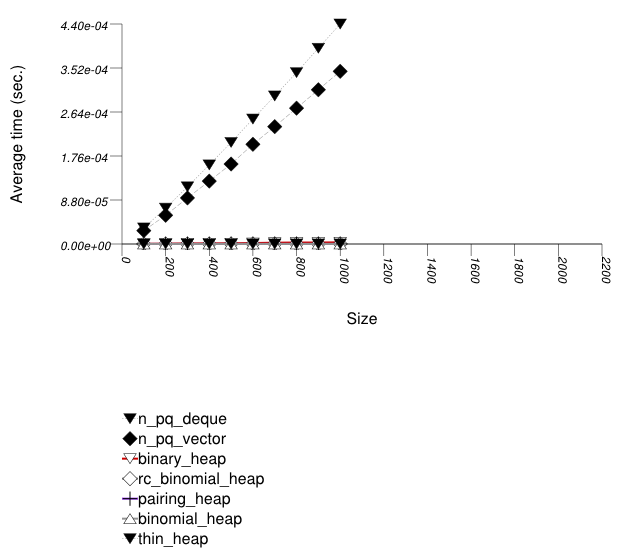The abbreviated names in the legend of the graphic above are instantiated with the types in the following table.

Name/Instantiating TypeParameterDetails
n_pq_vector
`std::priority_queue` `Sequence` `std::vector`
n_pq_deque
`std::priority_queue` `Sequence` `std::deque`
binary_heap
`priority_queue` `Tag` `binary_heap_tag`
binomial_heap
`priority_queue` `Tag` `binomial_heap_tag`
rc_binomial_heap
`priority_queue` `Tag` `rc_binomial_heap_tag`
thin_heap
`priority_queue` `Tag` `thin_heap_tag`
pairing_heap
`priority_queue` `Tag` `pairing_heap_tag`

The graphic below shows the results for the native priority queues and this library's pairing and thin heap priority_queue data structures.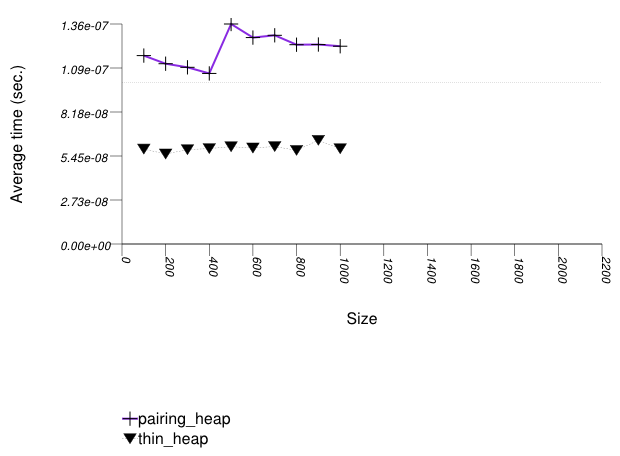The abbreviated names in the legend of the graphic above are instantiated with the types in the following table.

Name/Instantiating TypeParameterDetails
thin_heap
`priority_queue` `Tag` `thin_heap_tag`
pairing_heap
`priority_queue` `Tag` `pairing_heap_tag`
###### Observations

As noted above, increasing an arbitrary value (in the sense of the priority queue's comparison functor) is very common in graph-related algorithms. In this case, a thin heap (`priority_queue` with `Tag` = `thin_heap_tag`) outperforms a pairing heap (`priority_queue` with `Tag` = `pairing_heap_tag`). Conversely, Priority Queue Text `push` Timing Test, Priority Queue Text `push` and `pop` Timing Test, Priority Queue Random Integer `push` Timing Test, and Priority Queue Random Integer `push` and `pop` Timing Test show that the situation is reversed for other operations. It is not clear when to prefer one of these two different types.

In this test this library's binary heaps effectively perform modify in linear time. As explained in Priority Queue Design::Traits, given a valid point-type iterator, a binary heap can perform `modify` logarithmically. The problem is that binary heaps invalidate their find iterators with each modifying operation, and so the only way to obtain a valid point-type iterator is to iterate using a range-type iterator until finding the appropriate value, then use the range-type iterator for the `modify` operation.

The explanation for the standard's priority queues' performance is similar to that in Priority Queue Text `join` Timing Test.

##### Text `modify` Down

###### Description

This test inserts a number of values with keys from an arbitrary text ([ wickland96thirty ]) into into a container then modifies each one "down" (i.e., it makes it smaller). It uses `modify` for this library's priority queues; for the standard's priority queues, it pops values from a container until it reaches the value that should be modified, then pushes values back in. It measures the average time for `modify` as a function of the number of values.

It uses the test file: `performance/ext/pb_ds/priority_queue_text_modify_down_timing.cc`

The main purpose of this test is to contrast Priority Queue Text `modify` Up Timing Test.

###### Results

The two graphics below show the results for the native priority_queues and this library's priority_queues.

The graphic immediately below shows the results for the native priority_queue type instantiated with different underlying container types versus several different versions of library's priority_queues.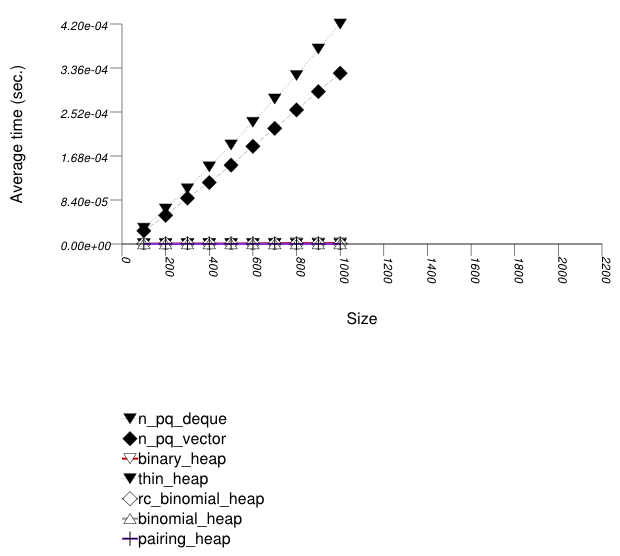The abbreviated names in the legend of the graphic above are instantiated with the types in the following table.

Name/Instantiating TypeParameterDetails
n_pq_vector
`std::priority_queue` `Sequence` `std::vector`
n_pq_deque
`std::priority_queue` `Sequence` `std::deque`
binary_heap
`priority_queue` `Tag` `binary_heap_tag`
binomial_heap
`priority_queue` `Tag` `binomial_heap_tag`
rc_binomial_heap
`priority_queue` `Tag` `rc_binomial_heap_tag`
thin_heap
`priority_queue` `Tag` `thin_heap_tag`
pairing_heap
`priority_queue` `Tag` `pairing_heap_tag`

The graphic below shows the results for the native priority queues and this library's pairing and thin heap priority_queue data structures.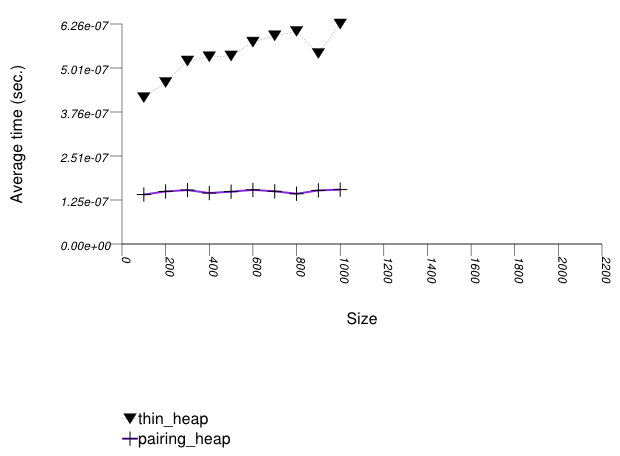The abbreviated names in the legend of the graphic above are instantiated with the types in the following table.

Name/Instantiating TypeParameterDetails
thin_heap
`priority_queue` `Tag` `thin_heap_tag`
pairing_heap
`priority_queue` `Tag` `pairing_heap_tag`
###### Observations

Most points in these results are similar to Priority Queue Text `modify` Up Timing Test.

It is interesting to note, however, that as opposed to that test, a thin heap (`priority_queue` with `Tag` = `thin_heap_tag`) is outperformed by a pairing heap (`priority_queue` with `Tag` = `pairing_heap_tag`). In this case, both heaps essentially perform an `erase` operation followed by a `push` operation. As the other tests show, a pairing heap is usually far more efficient than a thin heap, so this is not surprising.

Most algorithms that involve priority queues increase values (in the sense of the priority queue's comparison functor), and so Priority Queue Text `modify` Up Timing Test - is more interesting than this test.

#### Observations

##### Associative
###### Underlying Data-Structure Families

In general, hash-based containers have better timing performance than containers based on different underlying-data structures. The main reason to choose a tree-based or trie-based container is if a byproduct of the tree-like structure is required: either order-preservation, or the ability to utilize node invariants. If memory-use is the major factor, an ordered-vector tree gives optimal results (albeit with high modificiation costs), and a list-based container gives reasonable results.

###### Hash-Based Containers

Hash-based containers are typically either collision chaining or probing. Collision-chaining containers are more flexible internally, and so offer better timing performance. Probing containers, if used for simple value-types, manage memory more efficiently (they perform far fewer allocation-related calls). In general, therefore, a collision-chaining table should be used. A probing container, conversely, might be used efficiently for operations such as eliminating duplicates in a sequence, or counting the number of occurrences within a sequence. Probing containers might be more useful also in multithreaded applications where each thread manipulates a hash-based container: in the standard, allocators have class-wise semantics (see [meyers96more] - Item 10); a probing container might incur less contention in this case.

###### Hash Policies

In hash-based containers, the range-hashing scheme seems to affect performance more than other considerations. In most settings, a mask-based scheme works well (or can be made to work well). If the key-distribution can be estimated a-priori, a simple hash function can produce nearly uniform hash-value distribution. In many other cases (e.g., text hashing, floating-point hashing), the hash function is powerful enough to generate hash values with good uniformity properties [knuth98sorting]; a modulo-based scheme, taking into account all bits of the hash value, appears to overlap the hash function in its effort.

The range-hashing scheme determines many of the other policies. A mask-based scheme works well with an exponential-size policy; for probing-based containers, it goes well with a linear-probe function.

An orthogonal consideration is the trigger policy. This presents difficult tradeoffs. E.g., different load factors in a load-check trigger policy yield a space/amortized-cost tradeoff.

###### Branch-Based Containers

In general, there are several families of tree-based underlying data structures: balanced node-based trees (e.g., red-black or AVL trees), high-probability balanced node-based trees (e.g., random treaps or skip-lists), competitive node-based trees (e.g., splay trees), vector-based "trees", and tries. (Additionally, there are disk-residing or network-residing trees, such as B-Trees and their numerous variants. An interface for this would have to deal with the execution model and ACID guarantees; this is out of the scope of this library.) Following are some observations on their application to different settings.

Of the balanced node-based trees, this library includes a red-black tree, as does standard (in practice). This type of tree is the "workhorse" of tree-based containers: it offers both reasonable modification and reasonable lookup time. Unfortunately, this data structure stores a huge amount of metadata. Each node must contain, besides a value, three pointers and a boolean. This type might be avoided if space is at a premium [austern00noset].

High-probability balanced node-based trees suffer the drawbacks of deterministic balanced trees. Although they are fascinating data structures, preliminary tests with them showed their performance was worse than red-black trees. The library does not contain any such trees, therefore.

Competitive node-based trees have two drawbacks. They are usually somewhat unbalanced, and they perform a large number of comparisons. Balanced trees perform one comparison per each node they encounter on a search path; a splay tree performs two comparisons. If the keys are complex objects, e.g., `std::string`, this can increase the running time. Conversely, such trees do well when there is much locality of reference. It is difficult to determine in which case to prefer such trees over balanced trees. This library includes a splay tree.

Ordered-vector trees use very little space [austern00noset]. They do not have any other advantages (at least in this implementation).

Large-fan-out PATRICIA tries have excellent lookup performance, but they do so through maintaining, for each node, a miniature "hash-table". Their space efficiency is low, and their modification performance is bad. These tries might be used for semi-static settings, where order preservation is important. Alternatively, red-black trees cross-referenced with hash tables can be used. [okasaki98mereable] discusses small-fan-out PATRICIA tries for integers, but the cited results seem to indicate that the amortized cost of maintaining such trees is higher than that of balanced trees. Moderate-fan-out trees might be useful for sequences where each element has a limited number of choices, e.g., DNA strings.

###### Mapping-Semantics

Different mapping semantics were discussed in the introduction and design sections.Here the focus will be on the case where a keys can be composed into primary keys and secondary keys. (In the case where some keys are completely identical, it is trivial that one should use an associative container mapping values to size types.) In this case there are (at least) five possibilities:

1. Use an associative container that allows equivalent-key values (such as `std::multimap`)

2. Use a unique-key value associative container that maps each primary key to some complex associative container of secondary keys, say a tree-based or hash-based container.

3. Use a unique-key value associative container that maps each primary key to some simple associative container of secondary keys, say a list-based container.

4. Use a unique-key value associative container that maps each primary key to some non-associative container (e.g., `std::vector`)

5. Use a unique-key value associative container that takes into account both primary and secondary keys.

Stated simply: there is a simple answer for this. (Excluding option 1, which should be avoided in all cases).

If the expected ratio of secondary keys to primary keys is small, then 3 and 4 seem reasonable. Both types of secondary containers are relatively lightweight (in terms of memory use and construction time), and so creating an entire container object for each primary key is not too expensive. Option 4 might be preferable to option 3 if changing the secondary key of some primary key is frequent - one cannot modify an associative container's key, and the only possibility, therefore, is erasing the secondary key and inserting another one instead; a non-associative container, conversely, can support in-place modification. The actual cost of erasing a secondary key and inserting another one depends also on the allocator used for secondary associative-containers (The tests above used the standard allocator, but in practice one might choose to use, e.g., [boost_pool]). Option 2 is definitely an overkill in this case. Option 1 loses out either immediately (when there is one secondary key per primary key) or almost immediately after that. Option 5 has the same drawbacks as option 2, but it has the additional drawback that finding all values whose primary key is equivalent to some key, might be linear in the total number of values stored (for example, if using a hash-based container).

If the expected ratio of secondary keys to primary keys is large, then the answer is more complicated. It depends on the distribution of secondary keys to primary keys, the distribution of accesses according to primary keys, and the types of operations most frequent.

To be more precise, assume there are m primary keys, primary key i is mapped to ni secondary keys, and each primary key is mapped, on average, to n secondary keys (i.e., E(ni) = n).

Suppose one wants to find a specific pair of primary and secondary keys. Using 1 with a tree based container (`std::multimap`), the expected cost is E(Θ(log(m) + ni)) = Θ(log(m) + n); using 1 with a hash-based container (`std::tr1::unordered_multimap`), the expected cost is Θ(n). Using 2 with a primary hash-based container and secondary hash-based containers, the expected cost is O(1); using 2 with a primary tree-based container and secondary tree-based containers, the expected cost is (using the Jensen inequality [motwani95random]) E(O(log(m) + log(ni)) = O(log(m)) + E(O(log(ni)) = O(log(m)) + O(log(n)), assuming that primary keys are accessed equiprobably. 3 and 4 are similar to 1, but with lower constants. Using 5 with a hash-based container, the expected cost is O(1); using 5 with a tree based container, the cost is E(Θ(log(mn))) = Θ(log(m) + log(n)).

Suppose one needs the values whose primary key matches some given key. Using 1 with a hash-based container, the expected cost is Θ(n), but the values will not be ordered by secondary keys (which may or may not be required); using 1 with a tree-based container, the expected cost is Θ(log(m) + n), but with high constants; again the values will not be ordered by secondary keys. 2, 3, and 4 are similar to 1, but typically with lower constants (and, additionally, if one uses a tree-based container for secondary keys, they will be ordered). Using 5 with a hash-based container, the cost is Θ(mn).

Suppose one wants to assign to a primary key all secondary keys assigned to a different primary key. Using 1 with a hash-based container, the expected cost is Θ(n), but with very high constants; using 1 with a tree-based container, the cost is Θ(nlog(mn)). Using 2, 3, and 4, the expected cost is Θ(n), but typically with far lower costs than 1. 5 is similar to 1.

##### Priority_Queue
###### Complexity

The following table shows the complexities of the different underlying data structures in terms of orders of growth. It is interesting to note that this table implies something about the constants of the operations as well (see Amortized `push` and `pop` operations).

`push``pop``modify``erase``join`
`std::priority_queue` Θ(n) worst Θ(log(n)) amortized Θ(log(n)) Worst Θ(n log(n)) Worst [std note 1] Θ(n log(n)) [std note 2] Θ(n log(n)) [std note 1]
`priority_queue` <`Tag` = `pairing_heap_tag`> O(1) Θ(n) worst Θ(log(n)) amortized Θ(n) worst Θ(log(n)) amortized Θ(n) worst Θ(log(n)) amortized O(1)
`priority_queue` <`Tag` = `binary_heap_tag`> Θ(n) worst Θ(log(n)) amortized Θ(n) worst Θ(log(n)) amortized Θ(n) Θ(n) Θ(n)
`priority_queue` <`Tag` = `binomial_heap_tag`> Θ(log(n)) worst O(1) amortized Θ(log(n)) Θ(log(n)) Θ(log(n)) Θ(log(n))
`priority_queue` <`Tag` = `rc_binomial_heap_tag`> O(1) Θ(log(n)) Θ(log(n)) Θ(log(n)) Θ(log(n))
`priority_queue`<`Tag` = `thin_heap_tag`> O(1) Θ(n) worst Θ(log(n)) amortized Θ(log(n)) worst O(1) amortized, or Θ(log(n)) amortized [thin_heap_note] Θ(n) worst Θ(log(n)) amortized Θ(n)

[std note 1] This is not a property of the algorithm, but rather due to the fact that the standard's priority queue implementation does not support iterators (and consequently the ability to access a specific value inside it). If the priority queue is adapting an `std::vector`, then it is still possible to reduce this to Θ(n) by adapting over the standard's adapter and using the fact that `top` returns a reference to the first value; if, however, it is adapting an `std::deque`, then this is impossible.

[std note 2] As with [std note 1], this is not a property of the algorithm, but rather the standard's implementation. Again, if the priority queue is adapting an `std::vector` then it is possible to reduce this to Θ(n), but with a very high constant (one must call `std::make_heap` which is an expensive linear operation); if the priority queue is adapting an `std::deque`, then this is impossible.

[thin_heap_note] A thin heap has Θ(log(n)) worst case `modify` time always, but the amortized time depends on the nature of the operation: I) if the operation increases the key (in the sense of the priority queue's comparison functor), then the amortized time is O(1), but if II) it decreases it, then the amortized time is the same as the worst case time. Note that for most algorithms, I) is important and II) is not.

###### Amortized `push` and `pop` operations

In many cases, a priority queue is needed primarily for sequences of `push` and `pop` operations. All of the underlying data structures have the same amortized logarithmic complexity, but they differ in terms of constants.

The table above shows that the different data structures are "constrained" in some respects. In general, if a data structure has lower worst-case complexity than another, then it will perform slower in the amortized sense. Thus, for example a redundant-counter binomial heap (`priority_queue` with `Tag` = `rc_binomial_heap_tag`) has lower worst-case `push` performance than a binomial heap (`priority_queue` with `Tag` = `binomial_heap_tag`), and so its amortized `push` performance is slower in terms of constants.

As the table shows, the "least constrained" underlying data structures are binary heaps and pairing heaps. Consequently, it is not surprising that they perform best in terms of amortized constants.

1. Pairing heaps seem to perform best for non-primitive types (e.g., `std::string`s), as shown by Priority Queue Text `push` Timing Test and Priority Queue Text `push` and `pop` Timing Test

2. binary heaps seem to perform best for primitive types (e.g., ints), as shown by Priority Queue Random Integer `push` Timing Test and Priority Queue Random Integer `push` and `pop` Timing Test.

###### Graph Algorithms

In some graph algorithms, a decrease-key operation is required [clrs2001]; this operation is identical to `modify` if a value is increased (in the sense of the priority queue's comparison functor). The table above and Priority Queue Text `modify` Up Timing Test show that a thin heap (`priority_queue` with `Tag` = `thin_heap_tag`) outperforms a pairing heap (`priority_queue` with `Tag` = `Tag` = `pairing_heap_tag`), but the rest of the tests show otherwise.

This makes it difficult to decide which implementation to use in this case. Dijkstra's shortest-path algorithm, for example, requires Θ(n) `push` and `pop` operations (in the number of vertices), but O(n2) `modify` operations, which can be in practice Θ(n) as well. It is difficult to find an a-priori characterization of graphs in which the actual number of `modify` operations will dwarf the number of `push` and `pop` operations.